https://doi.org/10.5194/acp-20-12655-2020
https://doi.org/10.5194/acp-20-12655-2020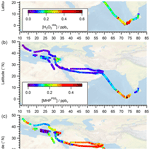# Impact of the South Asian monsoon outflow on atmospheric hydroperoxides in the upper troposphere

Bettina Hottmann, Sascha Hafermann, Laura Tomsche, Daniel Marno, Monica Martinez, Hartwig Harder, Andrea Pozzer, Marco Neumaier, Andreas Zahn, Birger Bohn, Greta Stratmann, Helmut Ziereis, Jos Lelieveld, and Horst Fischer
Abstract

During the OMO (Oxidation Mechanism Observation) mission, trace gas measurements were performed on board the HALO (High Altitude Long Range) research aircraft in summer 2015 in order to investigate the outflow of the South Asian summer monsoon and its influence on the composition of the Asian monsoon anticyclone (AMA) in the upper troposphere over the eastern Mediterranean and the Arabian Peninsula. This study focuses on in situ observations of hydrogen peroxide (${\mathrm{H}}_{\mathrm{2}}{{\mathrm{O}}_{\mathrm{2}}}^{\mathrm{obs}}$) and organic hydroperoxides (ROOHobs) as well as their precursors and loss processes. Observations are compared to photostationary-state (PSS) calculations of ${\mathrm{H}}_{\mathrm{2}}{{\mathrm{O}}_{\mathrm{2}}}^{\mathrm{PSS}}$ and extended by a separation of ROOHobs into methyl hydroperoxide (MHPPSS) and inferred unidentified hydroperoxide (UHPPSS) mixing ratios using PSS calculations. Measurements are also contrasted to simulations with the general circulation ECHAM–MESSy for Atmospheric Chemistry (EMAC) model. We observed enhanced mixing ratios of ${\mathrm{H}}_{\mathrm{2}}{{\mathrm{O}}_{\mathrm{2}}}^{\mathrm{obs}}$ (45 %), MHPPSS (9 %), and UHPPSS (136 %) in the AMA relative to the northern hemispheric background. Highest concentrations for ${\mathrm{H}}_{\mathrm{2}}{{\mathrm{O}}_{\mathrm{2}}}^{\mathrm{obs}}$ and MHPPSS of 211 and 152 ppbv, respectively, were found in the tropics outside the AMA, while for UHPPSS, with 208 pptv, highest concentrations were found within the AMA. In general, the observed concentrations are higher than steady-state calculations and EMAC simulations by a factor of 3 and 2, respectively. Especially in the AMA, EMAC underestimates the ${\mathrm{H}}_{\mathrm{2}}{{\mathrm{O}}_{\mathrm{2}}}^{\mathrm{EMAC}}$ (medians: 71 pptv vs. 164 pptv) and ROOHEMAC (medians: 25 pptv vs. 278 pptv) mixing ratios. Longitudinal gradients indicate a pool of hydroperoxides towards the center of the AMA, most likely associated with upwind convection over India. This indicates main contributions of atmospheric transport to the local budgets of hydroperoxides along the flight track, explaining strong deviations from steady-state calculations which only account for local photochemistry. Underestimation of ${\mathrm{H}}_{\mathrm{2}}{{\mathrm{O}}_{\mathrm{2}}}^{\mathrm{EMAC}}$ by approximately a factor of 2 in the Northern Hemisphere (NH) and the AMA and overestimation in the Southern Hemisphere (SH; factor 1.3) are most likely due to uncertainties in the scavenging efficiencies for individual hydroperoxides in deep convective transport to the upper troposphere, corroborated by a sensitivity study. It seems that the observed excess UHPPSS is excess MHP transported to the west from an upper tropospheric source related to convection in the summer monsoon over Southeast Asia.

Share
Dates
1 Introduction

The earth has an oxidizing atmosphere where OH functions as the main oxidizing agent (Levy, 1971). OH is formed by the photolysis of ozone (λ<320 nm) and subsequent reaction of the produced singlet D oxygen atom (O1D) with water vapor. The main sinks of OH are also the main sources of peroxy radicals (HO2 and RO2) in the reactions with CO, CH4, and volatile organic compounds (VOCs) and the reaction with nitrogen dioxide (NO2) to form nitric acid (HNO3). At low NOx (NO+NO2) concentrations, HO2 reacts with itself to form H2O2 or with RO2 to form organic hydroperoxides (ROOH). Since HO2, RO2, and especially CH3O2 react faster with NO than with HO2, peroxides are mainly produced in areas with low NO and high OH mixing ratios (Lee et al., 2000). H2O2 is a strong oxidant in the aqueous phase, oxidizing for example SO2 to H2SO4, and hence H2O2 partially contributes to acid rain formation (e.g., Hoffmann and Edwards, 1975; Penkett et al., 1979; Robbin Martin and Damschen, 1981; Calvert et al., 1985). The major photochemical sinks of hydroperoxides are photolysis, which recycles OH, and the reaction with OH forms HO2. Physical loss of hydroperoxides due to dry and wet deposition establishes an ultimate loss mechanism of HOx radicals. Thus H2O2 and ROOH play a pivotal role in the HOx budget and modulate the oxidation capacity of the atmosphere (Lelieveld and Crutzen, 1990; Crutzen et al., 1999).

The global distribution of hydroperoxides is affected by transport, physical removal by dry deposition and rainout, and net photochemical production processes. With increasing altitude – and thus decreasing water vapor concentration – the primary production of HOx decreases (Heikes et al., 1996) and leads to an increasing contribution of the photolysis of H2O2 and ROOH to the HOx budget (Jaeglé et al., 1997, 2000; Faloona et al., 2000, 2004). In more polluted areas, especially in the boundary layer, the H2O2 chemistry is more complex and leads to higher variabilities (Nunnermacker et al., 2008). Close to the surface, dry deposition of H2O2 forms a strong sink, resulting in decreasing concentrations with decreasing altitude. This often leads to a local maximum of H2O2 mixing ratios above the boundary layer at 2–5 km of altitude (Daum et al., 1990; Heikes, 1992; Weinstein-Lloyd et al., 1998; Snow, 2003; Snow et al., 2007; Klippel et al., 2011). A similar but weaker maximum at 2–5 km was found for methyl hydroperoxide (MHP; Weinstein-Lloyd et al., 1998; Snow, 2003; Snow et al., 2007). Due to its lower deposition velocity associated with less efficient uptake by solid and aqueous surfaces, MHP is not as sensitive to deposition processes as H2O2 (Lind and Kok, 1986, 1994), yielding rather constant mixing ratios with altitude within the boundary layer. Further, the mixing ratios of both species generally decrease with increasing latitude in the free troposphere due to lower water vapor concentrations (Jacob and Klockow, 1992; Perros, 1993; Slemr and Tremmel, 1994; Snow, 2003; Snow et al., 2007; Klippel et al., 2011).

In spite of several in situ measurement campaigns of trace gases in the outflow of the Asian summer monsoon in the recent years, e.g., from the IAGOS-CARIBIC (In-Service Aircraft for a Global Observing System – Civil Aircraft for the Regular Investigation of the atmosphere Based on an Instrument Container) project (Ojha et al., 2016; Rauthe-Schöch et al., 2016), the IAGOS-MOZAIC (Measurement of Ozone and Water Vapour on Airbus in-service Aircraft) project (Barret et al., 2016), the MINOS (Mediterranean Intensive Oxidant Study) aircraft campaign (Lelieveld et al., 2002), and the PEM-WEST (Pacific Exploratory Mission-West) A mission (Heikes et al., 1996), our understanding of the physical and chemical processes within the Asian monsoon anticyclone (AMA) is limited. So far we know that the updrafts of the summer monsoon deep convection can effectively transport insoluble pollutants from the surface to the upper troposphere, and there these polluted air masses can be transported over a long distance (Lawrence and Lelieveld, 2010). Thus the Asian summer monsoon has a strong influence on the upper troposphere (UT) and the lower stratosphere (Randel et al., 2010; Gettelman et al., 2004), and it is important to study its physical and chemical properties in greater detail.

The focus of the OMO (Oxidation Mechanism Observation) campaign was to investigate photochemical processes in the AMA in the UT. During the mission, the HALO (High Altitude and Long Range) research aircraft probed a large variety of air masses, ranging from clean Northern Hemisphere (NH) background air above the western Mediterranean to Southern Hemisphere (SH) background air over the northern Indian Ocean and air masses affected by the South Asian summer monsoon in the AMA over the Arabian Peninsula. The main goals of the campaign were to analyze the influence of the AMA on the oxidizing power of the atmosphere and to determine the rates at which natural and human-made compounds are converted by oxidation processes in the atmosphere (Lelieveld et al., 2018).

The present study addresses the budgets of H2O2 and organic hydroperoxides. Since the measurements of the sum of all organic hydroperoxides do not differentiate between different species, we estimate the contribution from MHPPSS based on steady-state calculations. In former studies MHP was identified as the most abundant organic hydroperoxide in the free troposphere (Heikes et al., 1996; Jackson and Hewitt, 1996). Our goal was to investigate the extent to which this is also the case for the outflow of the South Asian summer monsoon into the UT. In addition the in situ data were compared to results from the ECHAM–MESSy for Atmospheric Chemistry (EMAC) model (see Sect. 4.3.2) along the flight track for H2O2 and individual ROOH mixing ratios. ${\mathrm{H}}_{\mathrm{2}}{{\mathrm{O}}_{\mathrm{2}}}^{\mathrm{obs}}$ mixing ratios were also evaluated with steady-state calculations based on measured HOx and photolysis frequency measurements on board HALO.

2 The OMO project

The OMO campaign took place from 21 July to 27 August 2015. During the campaign 17 flights with the HALO research aircraft were performed. The airports of Oberpfaffenhofen (Germany), Paphos (Cyprus), Gan (Maldives), and Bahrain served as bases for takeoffs and landings. The flights were mainly performed over the Arabian Peninsula, the eastern Mediterranean, and the northern Indian Ocean (0.2 S–48.1 N, 11.3–80.2 E). In Fig. 1 the tracks of all OMO flights are shown. The aircraft reached altitudes up to 15 km, which corresponds to 130 hPa, to study the chemistry of the UT.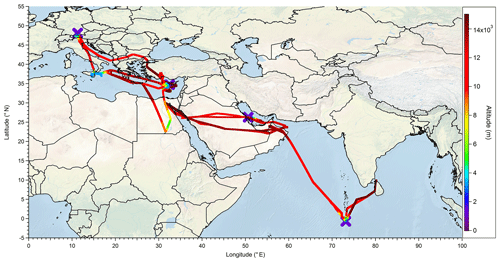Figure 1Flight tracks (colors indicate altitude) and airports (purple crosses) used during the OMO campaign.

3 Methods

## 3.1 Hydroperoxide measurements

The hydroperoxide data (${\mathrm{H}}_{\mathrm{2}}{{\mathrm{O}}_{\mathrm{2}}}^{\mathrm{obs}}$ and total organic hydroperoxides, ROOHobs) during OMO were obtained using a modified commercial instrument (AEROLASER, model AL2021, Garmisch-Partenkirchen, Germany) called HYPHOP (hydrogen peroxide and higher organic peroxide monitor). The HYPHOP instrument was installed in a 19′′ rack together with the infrared laser absorption instrument TRISTAR (tracer in situ TDLAS for atmospheric research) mounted in the back of HALO. Air was sampled from the top of the aircraft fuselage through a forward-facing trace gas inlet (TGI) designed as a bypass, consisting of a $\mathrm{1}/{\mathrm{2}}^{\prime \prime }$ PFA (perfluoroalkoxy alkane) tube inside the aircraft with an exit through a second TGI. From this bypass, air was sampled at a flow rate of 2 slpm (standard liters per minute) through a $\mathrm{1}/{\mathrm{4}}^{\prime \prime }$ PFA tube and directed to HYPHOP. To obtain constant pressure at the HYPHOP inlet, a constant pressure inlet (CPI) consisting of a dual-stage membrane pump (Vacuubrand MD1C VARIO SP, Wertheim, Germany) was used (Klippel et al., 2011).

HYPHOP relies on a dual-enzyme detection method after transfer of gaseous hydroperoxides into a buffered solution (potassium hydrogen phthalate and NaOH, pH 6) in a glass stripping coil (Lazrus et al., 1985, 1986). This stripper also contains EDTA (ethylenediaminetetraacetic acid) to prevent the reaction of transition metal ions with the hydroperoxides. Additionally, formaldehyde (HCHO) is added to prevent the oxidation of dissolved SO2 (in alkaline solutions ${\mathrm{HSO}}_{\mathrm{3}}^{-}$) by the hydroperoxides. Instead, HCHO and ${\mathrm{HSO}}_{\mathrm{3}}^{-}$ form hydroxymethyl sulfonate (${\mathrm{HOCH}}_{\mathrm{2}}{\mathrm{SO}}_{\mathrm{3}}^{-}$). After the stripping coil the hydroperoxide-containing solution is divided into two channels. Catalase is added to one channel in order to selectively destroy H2O2. This first channel thus measures only ROOH, while the second channel (without catalase) measures the sum of ROOH and H2O2. Since hydroperoxides cannot be detected by fluorescence directly, a second enzyme (horseradish peroxidase) and p-hydroxyphenylacetic acid (POPHA) are added to both channels. In a quantitative and selective reaction, the enzyme catalyzes the oxidation of POPHA by hydroperoxides, forming the fluorescent dye 6,6-dihydroxy-3,3-biphenyldiacetid acid. After excitation at 326 nm with a Cd lamp, the fluorescence at 400–420 nm is detected. To enlarge the fluorescence intensity, sodium hydroxide is added.

In order to perform zero measurements, the sampled air is directed through a cylinder filled with Hopcalite (MnO2 and CuO) to eliminate H2O2, ROOH, and ozone. Since the efficiency of Hopcalite decreases with increased humidity, the air is dried beforehand with the help of orange gel (SiO2 beads plus indicator).

To convert the detected signal into a concentration, a four-point calibration was performed before and after every flight. In the first two steps a liquid standard of H2O2 (1 µmol L−1; freshly diluted from stock solution) followed by zero air is measured in both channels without catalase. Afterwards this is repeated with catalase in the ROOH channel for the last two steps. The sensitivity for both channels and the catalase efficiency are determined via this procedure. The concentration of the liquid standard is based on titration of the stock solution (10 mmol L−1) with potassium permanganate.

To determine the stripping efficiency for H2O2, a gas-phase standard based on a permeation source (Teflon tube filled with 30 % H2O2 in a temperature-controlled glass flask) is used at a constant flow rate of approximately 40 sccm, diluted with synthetic air and measured with the instrument. The permeation rate of the source is quantified by collecting the output of the source into cooled water. The addition of hydrochloric titanium tetrachloride yields the formation of the yellow η2-peroxo complex $\left[\mathrm{Ti}\left({\mathit{\eta }}^{\mathrm{2}}-{\mathrm{O}}_{\mathrm{2}}\right){\mathrm{Cl}}_{\mathrm{4}}{\right]}^{\mathrm{2}-}$ (Pilz and Johann, 1974), whose concentration is determined via a UV photometer. The stripping efficiency of MHP was assumed to be 60% and that of H2O2 to be 100 % (AEROLASER, 2006; Lee et al., 2000).

The inlet efficiency was determined with the help of the permeation source, which was measured with and without the CPI. In laboratory studies the inlet efficiency was determined to be 87 % ± 3 %, decreasing during the campaign to 62.7 % ± 0.8 %, which is mainly due to the higher humidity.

The limit of detection (LOD) and precisions for H2O2 and MHP (assuming total ROOHobs to be only MHP), respectively, have been calculated for each flight from the reproducibility (1σ standard deviation) of in-flight zero (650 values) and liquid calibration (100 values) measurements, taking into account the sensitivity, stripping, and catalase efficiency. LOD values are in the range of 8–53 pptv for ${\mathrm{H}}_{\mathrm{2}}{{\mathrm{O}}_{\mathrm{2}}}^{\mathrm{obs}}$ (median 23 pptv) and 9–52 pptv for ROOHobs (median 23 pptv), respectively, assuming that ROOHobs is composed of MHP only. Please note that due to the fact that the exact composition of ROOHobs is unknown, and the solubility of different ROOH species can be quite variable, a detection limit for ROOHobs cannot be given. Instead, we calculate an upper limit of the detection limit, assuming that all ROOHobs consists of MHP, the species with the smallest solubility. Precision values were determined from the reproducibility of standard measurements and are in the range of 0.2 % at 5.2 ppbv and 1.3 % at 5.9 ppbv for H2O2 and 0.3 % at 5.0 ppbv and 2.1 % at 6.0 ppbv for MHP. The time resolution (signal increase from 10 % to 90 %) of the instrument is 120 s. An ozone interference of 53 pptv H2O2 per 100 ppbv O3, which was determined by H2O2 measurements in the stratosphere during the OMO-EU test campaign, was taken into account and corrected.

The total uncertainty calculated from statistical errors and uncertainties of liquid standard, inlet and stripping efficiency, and ozone interference is 25 % for H2O2 and 40 % for MHP.

## 3.2 Other in situ measurements

For this study CO, CH4, OH, HO2, O3, acetone, NO, NOy, ${J}_{{\mathrm{H}}_{\mathrm{2}}{\mathrm{O}}_{\mathrm{2}}}$, and JMHP data measured by other instruments have been used for data interpretation, steady-state calculations, and interference corrections (see Sect. 3.1). A complete list of all measured compounds can be found in Lelieveld et al. (2018). CO and CH4 have been measured by the IR quantum cascade laser absorption spectrometer TRISTAR (Schiller et al., 2008; Tadic et al., 2017). The measurements comprised an ambient-air mode and in-flight calibrations. The latter were realized with secondary standards from pressurized bottles (6 L bottle, Auer GmbH, Germany), which were calibrated against certified reference gases (Tomsche et al., 2019). With the help of the in-flight calibrations, the in situ data are drift-corrected by interpolation between two calibrations (Tadic et al., 2017). The observed CO and CH4 mixing ratios have a total uncertainty of 5.1 % and 0.275 %, respectively. The relatively high CO uncertainty reflects problems with the stability of the CO quantum cascade laser during the second half of OMO.

Laser-induced fluorescence was the method utilized for HOx measurements (instrument name: HORUS; Faloona et al., 2004; Martinez et al., 2010). The accuracies of the measurements are 17.1 % for OH and 17.6 % for HO2. The limit of detection of the instrument does vary depending on altitude as this system has a sensitivity that depends on pressure. As altitude increases the LOD decreases from 0.1 pptv to 0.02 pptv for OH and 0.361 to 0.175 pptv for HO2.

FAIRO (fast airborne ozone instrument) is a lightweight (14.5 kg) and accurate two-sensor device for measuring O3. It combines two techniques, i.e., (a) a UV photometer that measures the light absorption by O3 at a wavelength of λ= 250–260 nm emitted by a UV-LED and (b) a chemiluminescence detector that monitors the chemiluminescence generated by O3 on the surface of an organic dye adsorbed on dry silica gel. These techniques are simultaneously applied in order to combine the high measurement accuracy of the UV photometry with the high measurement frequency of the chemiluminescence detection. The UV photometer has a 1σ precision of 0.08 ppbv at a measurement frequency of 0.25 Hz (and a pressure of 1 bar) and an accuracy of 1.5 % (determined by the uncertainty of the O3 cross section). The chemiluminescence detector has a precision of 0.05 ppbv at a measurement frequency of 12.5 Hz (Zahn et al., 2012). In postprocessing the chemiluminescence detector data are calibrated using the UV photometer data.

Nitrogen oxide (NO) and total reactive nitrogen (NOy) were measured using the AENEAS atmospheric nitrogen oxide measuring system. The measurements were performed by a dual-channel NO chemiluminescence detector (CLD-SR 790, Eco Physics, Switzerland) in combination with a converter technique for the detection of total reactive nitrogen as NO. NOy comprises, among others, NO, NO2, HNO3, NO3, N2O5, HNO2, HO2NO2, PAN, and organic nitrates. The individual NOy species were detected after conversion to NO using a gold tube maintained at about 300 C, with H2 as a reducing agent (Ziereis et al., 2000). Ambient air was sampled using a standard HALO trace gas inlet equipped with a heated (∼40C) PFA inlet line. The time resolution of the measurements was about 1 s. The overall uncertainty of the NO and NOy measurements depends on its ambient concentrations and is about 8 % (6.5 %) for volume mixing ratios of 0.5 nmol mol−1 (1 nmol mol−1), respectively (Stratmann et al., 2016).

VOCs (e.g., acetone) were measured with a homebuilt lightweight (∼55 kg without rack) proton-transfer-reaction mass spectrometer, which uses a commercial quadrupole mass analyzer (Pfeiffer, QMA 410, Germany). A modular V25 microcomputer system (MPI-C, Mainz, Germany) is applied for instrument control and data acquisition. A custom-built inlet system comprises a platinum–quartz wool scrubber (Shimadzu, High Sensitivity Catalyst) held at 300 C and components for flow and pressure control. The instrument was calibrated between flights with a dynamically diluted gas standard containing approximately 500 ppbv of VOCs (Apel-Riemer Environmental Inc., USA). The accuracy for acetone is typically ±10 %, and the detection limit is ∼60 pptv.

Photolysis frequencies were calculated from spectral actinic flux density spectra (280–650 nm) obtained from charge-coupled device (CCD) spectroradiometer measurements on the top and bottom fuselage of the aircraft, covering the upper and the lower hemisphere, respectively (Bohn and Lohse, 2017). Recent recommendations of absorption cross sections and quantum yields were used in the calculations as well as their temperature and pressure dependencies (if available) by taking into account measured static air temperatures and pressures. Radiometric uncertainties range around 5 %–6 % under typical flight conditions. Additional uncertainties related to the molecular parameters are process-specific. For H2O2 in particular, recommended absorption cross sections and their temperature dependencies were applied, and unity quantum yields were assumed (Burkholder et al., 2015). However, the recommended H2O2 absorption cross sections are confined to a wavelength range below 350 nm, which is insufficient to capture atmospheric photolysis completely. Because measured cross sections decay exponentially over 2 orders of magnitude in the range of 280–350 nm, this dependence was further extrapolated up to 370 nm, where values drop well below 10−22 cm2. Dependent on conditions, this extrapolation increases atmospheric H2O2 photolysis frequencies by 10 %–20 %. For MHP the temperature dependence of the absorption cross sections is unknown. Therefore the recommended room temperature data were used under all conditions as well as unity quantum yields (Burkholder et al., 2015). Combined total uncertainties of 15 % and 25 % are estimated for H2O2 and MHP photolysis frequencies, respectively.

Latitude, longitude, and altitude data as well as temperature and pressure were collected with the BAHAMAS (basic HALO measurement and sensor system) instrument. More detailed information about the installation of scientific instruments and mission flights can be found at http://www.halo.dlr.de/science/missions/omo/omo.html (last access: 27 February 2019).

## 3.3 Photostationary-state calculations

Since only the sum of organic hydroperoxides was measured, we estimated the contribution of MHP using a photostationary-state (PSS) approximation relying on in situ measurements of HO2, OH, CO, CH4, NO, JMHP, and ${J}_{{\mathrm{H}}_{\mathrm{2}}{\mathrm{O}}_{\mathrm{2}}}$ (see Sect. 3.2) and rate coefficient data from Atkinson et al. (2004, 2006).

In the free troposphere the production rate P of H2O2 and MHP is due to the self-reaction of HO2 and reaction of CH3O2 with HO2, respectively, and can be calculated from Eqs. (1) and (2).

$\begin{array}{}\text{(1)}& P\left({\mathrm{H}}_{\mathrm{2}}{\mathrm{O}}_{\mathrm{2}}\right)={k}_{{\mathrm{HO}}_{\mathrm{2}}+{\mathrm{HO}}_{\mathrm{2}}}\cdot {\left[{\mathrm{HO}}_{\mathrm{2}}\right]}^{\mathrm{2}}\text{(2)}& P\left(\mathrm{MHP}\right)={k}_{{\mathrm{CH}}_{\mathrm{3}}{\mathrm{O}}_{\mathrm{2}}+{\mathrm{HO}}_{\mathrm{2}}}\cdot \left[{\mathrm{CH}}_{\mathrm{3}}{\mathrm{O}}_{\mathrm{2}}\right]\cdot \left[{\mathrm{HO}}_{\mathrm{2}}\right]\end{array}$

Photochemical loss rates L of H2O2 and MHP are due to photolysis and reaction with OH according to Eqs. (3) and (4).

$\begin{array}{}\text{(3)}& L\left({\mathrm{H}}_{\mathrm{2}}{\mathrm{O}}_{\mathrm{2}}\right)=\left({k}_{{\mathrm{H}}_{\mathrm{2}}{\mathrm{O}}_{\mathrm{2}}+\mathrm{OH}}\cdot \left[\mathrm{OH}\right]+{J}_{{\mathrm{H}}_{\mathrm{2}}{\mathrm{O}}_{\mathrm{2}}}\right)\cdot \left[{\mathrm{H}}_{\mathrm{2}}{\mathrm{O}}_{\mathrm{2}}\right]\text{(4)}& L\left(\mathrm{MHP}\right)=\left({k}_{\mathrm{MHP}+\mathrm{OH}}\cdot \left[\mathrm{OH}\right]+{J}_{\mathrm{MHP}}\right)\cdot \left[\mathrm{MHP}\right]\end{array}$

For steady-state conditions the production and loss reactions are at equilibrium, and the MHPPSS-to-${\mathrm{H}}_{\mathrm{2}}{{\mathrm{O}}_{\mathrm{2}}}^{\mathrm{obs}}$ ratio can be calculated from Eq. (5).

$\begin{array}{}\text{(5)}& \begin{array}{rl}\frac{{\left[\mathrm{MHP}\right]}^{\mathrm{PSS}}}{{\left[{\mathrm{H}}_{\mathrm{2}}{\mathrm{O}}_{\mathrm{2}}\right]}^{\mathrm{obs}}}& =\frac{{k}_{{\mathrm{CH}}_{\mathrm{3}}{\mathrm{O}}_{\mathrm{2}}+{\mathrm{HO}}_{\mathrm{2}}}\cdot \left[{\mathrm{CH}}_{\mathrm{3}}{\mathrm{O}}_{\mathrm{2}}\right]\cdot \left[{\mathrm{HO}}_{\mathrm{2}}\right]}{{k}_{{\mathrm{HO}}_{\mathrm{2}}+{\mathrm{HO}}_{\mathrm{2}}}\cdot {\left[{\mathrm{HO}}_{\mathrm{2}}\right]}^{\mathrm{2}}}\\ & \cdot \frac{{k}_{{\mathrm{H}}_{\mathrm{2}}{\mathrm{O}}_{\mathrm{2}}+\mathrm{OH}}\cdot \left[\mathrm{OH}\right]+{J}_{{\mathrm{H}}_{\mathrm{2}}{\mathrm{O}}_{\mathrm{2}}}}{{k}_{\mathrm{MHP}+\mathrm{OH}}\cdot \left[\mathrm{OH}\right]+{J}_{\mathrm{MHP}}}\end{array}\end{array}$

Because individual peroxy radicals were not measured, the CH3O2-to-HO2 ratio must be estimated from their production and loss terms. This ratio can be deduced as written in Eq. (6).

$\begin{array}{}\text{(6)}& \frac{\left[{\mathrm{CH}}_{\mathrm{3}}{\mathrm{O}}_{\mathrm{2}}\right]}{\left[{\mathrm{HO}}_{\mathrm{2}}\right]}=\frac{L\left({\mathrm{HO}}_{\mathrm{2}}\right)\cdot P\left({\mathrm{CH}}_{\mathrm{3}}{\mathrm{O}}_{\mathrm{2}}\right)}{P\left({\mathrm{HO}}_{\mathrm{2}}\right)\cdot L\left({\mathrm{CH}}_{\mathrm{3}}{\mathrm{O}}_{\mathrm{2}}\right)}\end{array}$

Dominant loss processes for HO2 and CH3O2 are reactions with NO and the production of H2O2 and MHP, respectively, neglecting the production of peroxy nitrates due to low NO2 concentrations in the UT (Eqs. 7 and 8).

$\begin{array}{}\text{(7)}& \begin{array}{rl}L\left({\mathrm{HO}}_{\mathrm{2}}\right)& ={k}_{{\mathrm{CH}}_{\mathrm{3}}{\mathrm{O}}_{\mathrm{2}}+{\mathrm{HO}}_{\mathrm{2}}}\cdot \left[{\mathrm{CH}}_{\mathrm{3}}{\mathrm{O}}_{\mathrm{2}}\right]\cdot \left[{\mathrm{HO}}_{\mathrm{2}}\right]+{k}_{{\mathrm{HO}}_{\mathrm{2}}+\mathrm{NO}}\\ & \cdot \left[{\mathrm{HO}}_{\mathrm{2}}\right]\cdot \left[\mathrm{NO}\right]+{k}_{{\mathrm{HO}}_{\mathrm{2}}+{\mathrm{HO}}_{\mathrm{2}}}\cdot {\left[{\mathrm{HO}}_{\mathrm{2}}\right]}^{\mathrm{2}}\end{array}\text{(8)}& \begin{array}{rl}L\left({\mathrm{CH}}_{\mathrm{3}}{\mathrm{O}}_{\mathrm{2}}\right)& ={k}_{{\mathrm{CH}}_{\mathrm{3}}{\mathrm{O}}_{\mathrm{2}}+{\mathrm{HO}}_{\mathrm{2}}}\cdot \left[{\mathrm{CH}}_{\mathrm{3}}{\mathrm{O}}_{\mathrm{2}}\right]\cdot \left[{\mathrm{HO}}_{\mathrm{2}}\right]\\ & +{k}_{{\mathrm{CH}}_{\mathrm{3}}{\mathrm{O}}_{\mathrm{2}}+\mathrm{NO}}\cdot \left[{\mathrm{CH}}_{\mathrm{3}}{\mathrm{O}}_{\mathrm{2}}\right]\cdot \left[\mathrm{NO}\right]\end{array}\end{array}$

The first terms on the right side of both equations are identical. The second terms are dominated by the rate coefficients of the reactions with NO and the NO concentration. For the calculations of the rate coefficients, the mean temperature of 259.18 K, the mean altitude of 10 992.8 m, and the mean pressure of 22 932.9 Pa were used. The resulting values are shown in Eqs. (9)–(11). As the relative humidity is very low in the upper troposphere, the water dependence in Eq. (11) was neglected.

$\begin{array}{}\text{(9)}& \begin{array}{rl}{k}_{{\mathrm{HO}}_{\mathrm{2}}+\mathrm{NO}}& =\mathrm{3.45}×{\mathrm{10}}^{-\mathrm{12}}\cdot {\mathrm{exp}}^{\frac{\mathrm{270}}{T}}\\ & =\mathrm{9.78}×{\mathrm{10}}^{-\mathrm{12}}{\mathrm{cm}}^{\mathrm{3}}\phantom{\rule{0.125em}{0ex}}\left(\mathrm{molec}.\phantom{\rule{0.125em}{0ex}}\mathrm{s}{\right)}^{-\mathrm{1}}\end{array}\text{(10)}& \begin{array}{rl}{k}_{{\mathrm{CH}}_{\mathrm{3}}{\mathrm{O}}_{\mathrm{2}}+\mathrm{NO}}& =\mathrm{2.3}×{\mathrm{10}}^{-\mathrm{12}}\cdot {\mathrm{exp}}^{\frac{\mathrm{360}}{T}}\\ & =\mathrm{9.22}×{\mathrm{10}}^{-\mathrm{12}}\phantom{\rule{0.125em}{0ex}}{\mathrm{cm}}^{\mathrm{3}}\phantom{\rule{0.125em}{0ex}}\left(\mathrm{molec}.\phantom{\rule{0.125em}{0ex}}\mathrm{s}{\right)}^{-\mathrm{1}}\end{array}\text{(11)}& \begin{array}{rl}{k}_{{\mathrm{HO}}_{\mathrm{2}}+{\mathrm{HO}}_{\mathrm{2}}}& =\mathrm{2.2}×{\mathrm{10}}^{-\mathrm{13}}\cdot {\mathrm{exp}}^{\frac{\mathrm{600}}{T}}\\ & +\mathrm{1.9}×{\mathrm{10}}^{-\mathrm{33}}\cdot \left[{\mathrm{N}}_{\mathrm{2}}\right]\cdot {\mathrm{exp}}^{\frac{\mathrm{980}}{T}}\\ & =\mathrm{2.64}×{\mathrm{10}}^{-\mathrm{12}}\phantom{\rule{0.125em}{0ex}}{\mathrm{cm}}^{\mathrm{3}}\phantom{\rule{0.125em}{0ex}}\left(\mathrm{molec}.\phantom{\rule{0.125em}{0ex}}\mathrm{s}{\right)}^{-\mathrm{1}}\end{array}\end{array}$

This indicates that the reaction of HO2 with NO is more than a factor of 3 faster than the self-reaction. The measured NO concentration is an order of magnitude larger than measured HO2 so that reaction with NO is the dominant process for both HO2 and CH3O2, resulting in similar loss rates for both radicals in the UT. Thus, the ratio of CH3O2 to HO2 is dominated by their production rates (Eq. 12).

$\begin{array}{}\text{(12)}& \frac{\left[{\mathrm{CH}}_{\mathrm{3}}{\mathrm{O}}_{\mathrm{2}}\right]}{\left[{\mathrm{HO}}_{\mathrm{2}}\right]}=\frac{P\left({\mathrm{CH}}_{\mathrm{3}}{\mathrm{O}}_{\mathrm{2}}\right)}{P\left({\mathrm{HO}}_{\mathrm{2}}\right)}=\frac{{k}_{{\mathrm{CH}}_{\mathrm{4}}+\mathrm{OH}}\cdot \left[{\mathrm{CH}}_{\mathrm{4}}\right]\cdot \left[\mathrm{OH}\right]}{{k}_{\mathrm{CO}+\mathrm{OH}}\cdot \left[\mathrm{CO}\right]\cdot \left[\mathrm{OH}\right]}\end{array}$

The combination of Eqs. (5) and (12) results in Eq. (13), which was used to calculate the MHPPSS concentrations based on the observed mixing ratios during OMO.

$\begin{array}{}\text{(13)}& \begin{array}{rl}{\left[\mathrm{MHP}\right]}^{\mathrm{PSS}}& =\frac{{k}_{{\mathrm{CH}}_{\mathrm{3}}{\mathrm{O}}_{\mathrm{2}}+{\mathrm{HO}}_{\mathrm{2}}}}{{k}_{{\mathrm{HO}}_{\mathrm{2}}+{\mathrm{HO}}_{\mathrm{2}}}}\\ & \cdot \frac{{k}_{{\mathrm{H}}_{\mathrm{2}}{\mathrm{O}}_{\mathrm{2}}+\mathrm{OH}}\cdot {\left[\mathrm{OH}\right]}^{\mathrm{obs}}+{J}_{{\mathrm{H}}_{\mathrm{2}}{\mathrm{O}}_{\mathrm{2}}}^{\mathrm{obs}}}{{k}_{\mathrm{MHP}+\mathrm{OH}}\cdot }\cdot {\left[\mathrm{OH}\right]}^{\mathrm{obs}}\\ & +{J}_{\mathrm{MHP}}^{\mathrm{obs}}\cdot \frac{{k}_{{\mathrm{CH}}_{\mathrm{4}}+\mathrm{OH}}\cdot {\left[{\mathrm{CH}}_{\mathrm{4}}\right]}^{\mathrm{obs}}}{{k}_{\mathrm{CO}+\mathrm{OH}}\cdot {\left[\mathrm{CO}\right]}^{\mathrm{obs}}}\cdot {\left[{\mathrm{H}}_{\mathrm{2}}{\mathrm{O}}_{\mathrm{2}}\right]}^{\mathrm{obs}}\end{array}\end{array}$

Please note that other sources of HO2 and CH3O2, in particular the photolysis of formaldehyde (HCHO) and acetaldehyde, respectively have been neglected. This is justified by the generally low mixing ratios of these species at high altitudes. Measurements of HCHO with the TRISTAR instrument yielded values below the detection limit of 30 pptv (parts per trillion by volume), and although acetaldehyde was not measured, we assume that its mixing ratio is within a factor of 2 of those for HCHO.

The total uncertainty of MHPPSS from the calculation according to Eq. (13) can be deduced from error propagation, taking into account uncertainties in OHobs (17.1 %), ${J}_{{\mathrm{H}}_{\mathrm{2}}{\mathrm{O}}_{\mathrm{2}}}^{\mathrm{obs}}$ (15 %), ${J}_{\mathrm{MHP}}^{\mathrm{obs}}$ (25 %), ${\mathrm{CH}}_{\mathrm{4}}^{\mathrm{obs}}$ (0.275 %), COobs (5.1 %), ${\mathrm{H}}_{\mathrm{2}}{{\mathrm{O}}_{\mathrm{2}}}^{\mathrm{obs}}$ (25 %), and rate constants, to be of the order of 45 % (1σ).

To estimate the contribution of MHP to the total organic hydroperoxides, the calculated concentration of MHPPSS was subtracted from the measured sum of all organic hydroperoxides ROOHobs. This leads to a concentration of unidentified organic hydroperoxides (UHPPSS; Eq. 14). Please note that MHPPSS only accounts for local CH4 oxidation production and not for transport phenomena.

$\begin{array}{}\text{(14)}& {\left[\mathrm{UHP}\right]}^{\mathrm{PSS}}={\left[\mathrm{ROOH}\right]}^{\mathrm{obs}}-\left[\mathrm{MHP}{\right]}^{\mathrm{PSS}}\end{array}$

## 3.4H2O2 calculation

In order to classify the measured ${\mathrm{H}}_{\mathrm{2}}{{\mathrm{O}}_{\mathrm{2}}}^{\mathrm{obs}}$, ${{\mathrm{HO}}_{\mathrm{2}}}^{\mathrm{obs}}$, and OHobs data, we calculated ${\mathrm{H}}_{\mathrm{2}}{{\mathrm{O}}_{\mathrm{2}}}^{\mathrm{PSS}}$ from measured HOx and ${J}_{{\mathrm{H}}_{\mathrm{2}}{\mathrm{O}}_{\mathrm{2}}}^{\mathrm{obs}}$. For the calculation, Eq. (15) was used, which is based on Eqs. (1) and (3).

$\begin{array}{}\text{(15)}& {\left[{\mathrm{H}}_{\mathrm{2}}{\mathrm{O}}_{\mathrm{2}}\right]}^{\mathrm{PSS}}=\frac{{\left[{\mathrm{HO}}_{\mathrm{2}}\right]}^{\mathrm{obs}\phantom{\rule{0.125em}{0ex}}\mathrm{2}}\cdot {k}_{{\mathrm{HO}}_{\mathrm{2}}+{\mathrm{HO}}_{\mathrm{2}}}}{{\left[\mathrm{OH}\right]}^{\mathrm{obs}}\cdot {k}_{{\mathrm{H}}_{\mathrm{2}}{\mathrm{O}}_{\mathrm{2}}+\mathrm{OH}}+{J}_{{\mathrm{H}}_{\mathrm{2}}{\mathrm{O}}_{\mathrm{2}}}^{\mathrm{obs}}}\end{array}$

A total uncertainty of 45 % (1σ) due to uncertainties in OHobs (17.1 %), ${{\mathrm{HO}}_{\mathrm{2}}}^{\mathrm{obs}}$ (17.6 %), ${J}_{{\mathrm{H}}_{\mathrm{2}}{\mathrm{O}}_{\mathrm{2}}}^{\mathrm{obs}}$ (15 %), and reaction rate constants was calculated.

## 3.5 Other research tools

The EMAC (ECHAM–MESSy atmospheric chemistry) model comprises the fifth generation of the European Center Hamburg (ECHAM5; Roeckner et al., 2006; version 5.3.01) general circulation model and the Modular Earth Submodel System (MESSy; Jöckel et al., 2016; version 2.52, http://www.messy-interface.org/, last access: 25 August 2019). For this study EMAC simulations (T42L90, 2.8× 2.8 horizontal resolution, 90 vertical levels to 0.01 hPa, time resolution 12 min) were sampled along the OMO flights tracks. Detailed specifications and results have been published previously (Lelieveld et al., 2018; Tomsche et al., 2019).

Back trajectories of 10 d were calculated along the flight path using FLEXPART to identify the air mass origin (Tomsche et al., 2019). Convective transport can be simulated in FLEXPART with the convection parameterization by Emanuel and Zivkovic-Rothman (1999). To represent moist convection realistically in models, the parametrization includes cloud microphysical processes, the physics of entrainment and mixing, and large-scale control of ensemble convective activity. It builds on temperature and humidity fields to provide mass flux information (Stohl et al., 2005). The back trajectories in the present paper are calculated with the convective parametrization. Further, the Lagrangian particle dispersion model FLEXPART produces so-called centroid trajectories based on the analysis of a cluster of trajectories. These trajectories are comparable to traditional trajectories but include convection via the centroid of all particles per time step. As indicated by Tomsche et al. (2019), prominent source regions of AMA air masses are identified to be the Indo-Gangetic Plain, northeastern India, Bangladesh, and the Bay of Bengal. Additionally, Tomsche et al. used observations of methane to differentiate between air masses influenced by the AMA and background air. A comparison of vertical profiles indicated that the air inside the AMA showed significantly higher CH4 concentrations than outside. Thus a threshold of CH4≥1879.8 ppbv was used to distinguish between air masses influenced by the AMA (CH4≥1879.8 ppbv), the SH background (CH4<1820 ppbv), and the NH background (1820 ppb${}_{\mathrm{v}}\le {\mathrm{CH}}_{\mathrm{4}}<\mathrm{1879.8}$ ppbv; Tomsche et al., 2019).

4 Results and discussion

## 4.1 Data processing

Data were collected from a merged data set given as 60 s means (calculated from the original data set obtained at higher resolutions) in order to get the same time resolution for all compounds. The given time is the middle of the block mean.

For the histograms the concentrations of all species shown were binned into samples with a width of 10 pptv, starting with the plots with the lowest bin. To compare the simulations from EMAC with measured and PSS-calculated data, the corresponding values (out of the 60 s means) were used at the given times from EMAC.

## 4.2 Case study: Flight 17 from Gan to Bahrain (10 August 2015)

In a case study analyzing Flight 17 from 10 August 2015, the method used to determine the origin of the measured air masses and a quantification and comparison of measured and simulated mixing ratios of ${\mathrm{H}}_{\mathrm{2}}{{\mathrm{O}}_{\mathrm{2}}}^{\mathrm{obs}}$, MHPPSS, and UHPPSS is presented. During this flight we encountered the SH and NH as well as the AMA in the UT. The flight track is shown in Fig. 2 (dotted line). Takeoff was in Gan (Maldives) and landing in Bahrain, on the Arabian Peninsula. Besides takeoff and landing, the entire flight took place in the UT (<230 hPa). The calculated back trajectories show the origin of the air masses. At the beginning of the flight the measured air masses had their origin over the Indian Ocean and Indonesia. During the remaining flight the measured air stemmed from India. Tomsche et al. (2019) showed that the measured air in the AMA was affected by deep convection over India, resulting in methane mixing ratios above the threshold. Figure 3 shows the time series for ${\mathrm{H}}_{\mathrm{2}}{{\mathrm{O}}_{\mathrm{2}}}^{\mathrm{obs}}$ during the flight at the time steps given from the frequency of EMAC output (orange circles). The colored bar on top shows the origin of air masses, i.e., red for AMA, green for NH, and blue for SH. The ${\mathrm{H}}_{\mathrm{2}}{{\mathrm{O}}_{\mathrm{2}}}^{\mathrm{obs}}$ mixing ratios vary between 128 and 366 pptv. The modeled ${\mathrm{H}}_{\mathrm{2}}{{\mathrm{O}}_{\mathrm{2}}}^{\mathrm{EMAC}}$ data are in the range of 110–799 pptv (gray triangles). For the beginning of the flight, model simulations agree rather well with the measurement data. At around 06:00 UTC the model-calculated mixing ratios increase to more than 500 pptv, while the measured data decrease to 200 pptv and lower. In this period a maximum difference between model and in situ data of 386 pptv was found. After 1 h the EMAC model data decrease to 416 pptv, and the in situ data increase to 214 pptv. During the following hour until around 08:00 UTC and thus at higher altitude, both mixing ratios increase, with the modeled data showing a much stronger increase up to approximately 800 pptv, while the in situ data increase only to 230 pptv. During the last period of the flight, simulated and measured data are again in good agreement. Here the mixing ratios from EMAC are in the range of 110–157 pptv, while the measured data are in the range of 128–203 pptv. This steep drop of ${\mathrm{H}}_{\mathrm{2}}{{\mathrm{O}}_{\mathrm{2}}}^{\mathrm{EMAC}}$ might arise due to a change in the flight altitude since between 08:01 and 08:06 UTC the aircraft changed from a flight level at 11 700 m to one aloft at 13 900 m.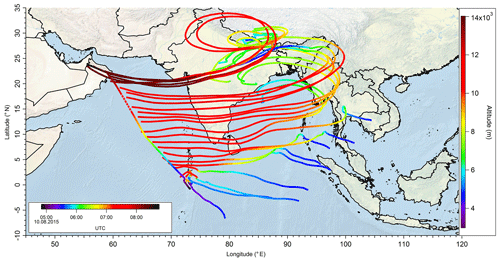Figure 2Track of Flight 17 (dotted black line) and calculated 10 d back trajectories (lines colored as a function of altitude) to show the origin of sampled air masses during the flight.

In addition to EMAC simulations, Fig. 3 also shows the calculated ${\mathrm{H}}_{\mathrm{2}}{{\mathrm{O}}_{\mathrm{2}}}^{\mathrm{PSS}}$ obtained from Eq. (15). Observed and PSS values for H2O2 mixing ratios agree very well, with a median deviation of 42 pptv, well within the combined uncertainties of measured data (25 %) and PSS simulations (45 %).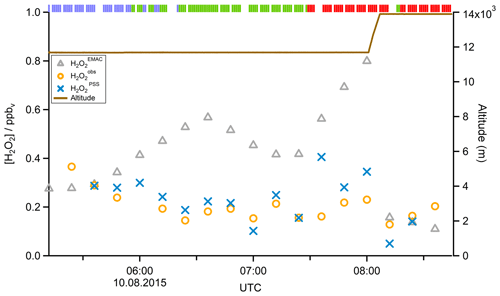Figure 3Time series of ${\mathrm{H}}_{\mathrm{2}}{{\mathrm{O}}_{\mathrm{2}}}^{\mathrm{obs}}$ (orange circles), ${\mathrm{H}}_{\mathrm{2}}{{\mathrm{O}}_{\mathrm{2}}}^{\mathrm{PSS}}$ (blue crosses), and ${\mathrm{H}}_{\mathrm{2}}{{\mathrm{O}}_{\mathrm{2}}}^{\mathrm{EMAC}}$ (gray triangles) mixing ratios for Flight 17. The brown line shows the altitude; the colored bar on top indicates the origin of air masses according to the methane mixing ratio classification: for SH blue, NH green, and AMA red.

In Fig. 4 the time series of ${\mathrm{H}}_{\mathrm{2}}{{\mathrm{O}}_{\mathrm{2}}}^{\mathrm{obs}}$, MHPPSS, and UHPPSS mixing ratios are shown (5 min means). In the beginning of the flight, MHPPSS is the dominant organic hydroperoxide. The mixing ratios are in the range of 140–341 pptv, similar to those of ${\mathrm{H}}_{\mathrm{2}}{{\mathrm{O}}_{\mathrm{2}}}^{\mathrm{obs}}$ (143–337 pptv). UHPPSS mixing ratios are in the range of 24–162 pptv, with a mean of 89 pptv. Later during the flight, UHPPSS are the dominant hydroperoxides in air masses inside the AMA originating from India. Here we found UHPPSS mixing ratios up to 275 pptv. The ${\mathrm{H}}_{\mathrm{2}}{{\mathrm{O}}_{\mathrm{2}}}^{\mathrm{obs}}$ mixing ratios show a similar temporal pattern and mixing ratio levels to those of UHPPSS over the Arabian Sea and the Arabian Peninsula, with values in the range of 140–243 pptv. MHPPSS mixing ratios are much lower (62–130 pptv, median of 72 pptv) in this area. During this part of the flight, the similarity in the time series of acetone and UHPPSS (Fig. 5) indicates either similar source regions for both species or the role of acetone as a precursor for the UHPPSS in AMA-influenced air masses. From 06:20 UTC onwards an increase in both compounds is observed until 07:45 UTC, followed by a steep drop, with a minimum at 08:17 UTC. The UHPPSS and acetone mixing ratios in this part of the flight are strongly correlated (Fig. 6), with a slope of 0.19±0.02 (ppbv ppbv−1) and an offset of ($-\mathrm{0.003}±\mathrm{0.02}$) ppbv. The regression coefficient R2 is very high (0.82). For ${\mathrm{H}}_{\mathrm{2}}{{\mathrm{O}}_{\mathrm{2}}}^{\mathrm{obs}}$, MHPPSS, and ROOHobs the correlation is not that strong, with slopes of $-\mathrm{0.02}±\mathrm{0.02}$ (ppbv ppbv−1), $-\mathrm{0.07}±\mathrm{0.01}$ (ppbv ppbv−1), and 0.13±0.03 (ppbv ppbv−1), respectively, and offsets of (0.21±0.02) ppbv, (0.12±0.01) ppbv, and (0.11±0.03) ppbv (Fig. 6). The relation between ROOH mixing ratios and an air mass age tracer based on the ratio between [NO] and [NOy] shows higher values of ROOH at smaller ratios, representing older or more processed air masses (Fig. 7) since the highest ROOH mixing ratios (>200 pptv) are found at the lowest [NO]  [NOy] ratios (all <0.19). Thus, most of the observed ROOH were measured in aged air masses transported within the anticyclone. The correlation with UHPPSS shows that this effect is mainly due to UHPPSS. For ${\mathrm{H}}_{\mathrm{2}}{{\mathrm{O}}_{\mathrm{2}}}^{\mathrm{obs}}$ there are also some higher mixing ratios for high [NO]-to-[NOy] mixing ratios and thus fresher air (Fig. 7).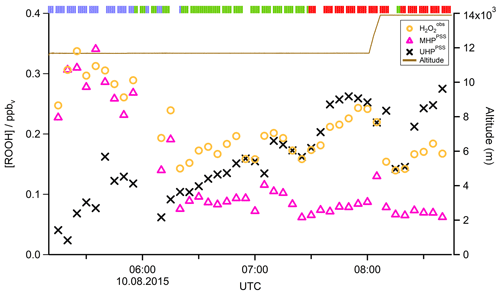Figure 4Time series of hydroperoxide mixing ratios during Flight 17. The mixing ratios of ${\mathrm{H}}_{\mathrm{2}}{{\mathrm{O}}_{\mathrm{2}}}^{\mathrm{obs}}$ (orange circles), MHPPSS (pink triangles), and UHPPSS (black crosses) are shown. The brown line shows the altitude; the colored bar on top indicates the origin of air masses according to the methane mixing ratio classification: for SH blue, NH green, and AMA red.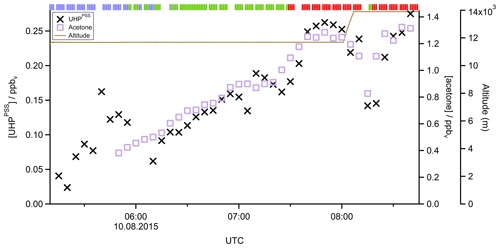Figure 5Time series of UHPPSS (black crosses) and acetone (purple squares) mixing ratios during Flight 17. The brown line shows the altitude, the colored bar on top indicates the origin of air masses according to the methane mixing ratio classification: for SH blue, NH green, and AMA red.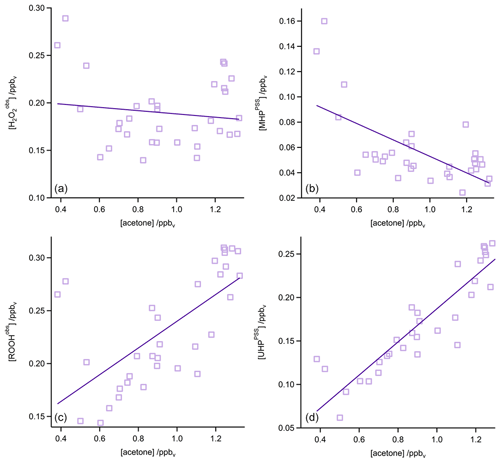Figure 6Scatterplots of measured acetone and ${\mathrm{H}}_{\mathrm{2}}{{\mathrm{O}}_{\mathrm{2}}}^{\mathrm{obs}}$ (a), MHPPSS (b), ROOHobs (c), and UHPPSS (d) during Flight 17. The dark purple lines represent the least-orthogonal-distance fits with regression coefficients R2 of 0.02, 0.41, 0.43, and 0.82.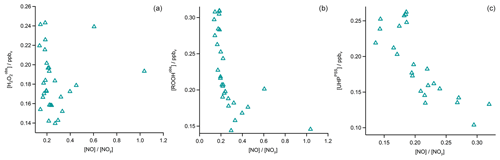Figure 7Scatterplots of ${\mathrm{H}}_{\mathrm{2}}{{\mathrm{O}}_{\mathrm{2}}}^{\mathrm{obs}}$ (a), ROOHobs (b), and UHPPSS (c) and NO∕NOy ratio during Flight 17.

## 4.3 Results for the entire campaign

To extend the analysis to the entire campaign, Fig. 8 shows all flight tracks in the UT during OMO. The color code represents observed mixing ratios of ${\mathrm{H}}_{\mathrm{2}}{{\mathrm{O}}_{\mathrm{2}}}^{\mathrm{obs}}$, MHPPSS, and UHPPSS varying from low (purple) to high values (red). Histograms for the whole campaign of ${\mathrm{H}}_{\mathrm{2}}{{\mathrm{O}}_{\mathrm{2}}}^{\mathrm{obs}}$ mixing ratios as well as inferred MHPPSS and UHPPSS mixing ratios are presented in Fig. 9. Here only data from the UT (<300 hPa, which corresponds to altitudes >9 km) were included in the analysis. Mixing ratios for all species were further differentiated by methane levels such that data in air masses with CH4 mixing ratios above the threshold of 1879.8 ppbv were classified as AMA-influenced, while air masses with CH4 mixing ratios between 1820 ppbv and 1879.8 ppbv were classified as NH background and those with CH4<1820 ppbv as SH following Tomsche et al. (2019). Figure 9a indicates that ${\mathrm{H}}_{\mathrm{2}}{{\mathrm{O}}_{\mathrm{2}}}^{\mathrm{obs}}$ mixing ratios are most abundant at values of 70–90 pptv in NH background air masses (green); 130 to 270 pptv with three notable peaks at 180–190, 210–220, and 250–270 pptv in SH air masses (blue); and 150–170 pptv in AMA-influenced air masses (red). The medians are 115 pptv for the NH background, 211 pptv for the SH, and 167 pptv for the AMA, indicating an excess of 52 pptv in AMA-influenced air masses compared to the NH background, while in the SH the ${\mathrm{H}}_{\mathrm{2}}{{\mathrm{O}}_{\mathrm{2}}}^{\mathrm{obs}}$ mixing ratio is twice as high as the NH background. For MHPPSS (Fig. 9b) the frequency distribution in the NH background shows a maximum at 30–40 pptv (green). For AMA-influenced air a sharp maximum at 50–70 pptv (red) is found. Air masses from the SH exhibit a rather flat distribution, with a maximum at values of 40–50 pptv and a median of 152 pptv (blue). With median mixing ratios of 70 and 64 pptv, we found only slightly higher mixing ratios for AMA-influenced air masses in comparison to the NH background. For UHPPSS (Fig. 9c) we again found a flat distribution of mixing ratios in the SH (blue), with a maximum at values of 140–150 pptv and a median of 129 pptv. The maximum in the frequency distribution for NH background conditions is found at 70–90 pptv (green), while in AMA-influenced air masses it was significantly higher, with 210–230 pptv (red). Thus, mixing ratios of UHPPSS are approximately 2–3 times higher in the AMA outflow than in the NH background. This is also represented in the medians of 210 pptv in the AMA and 89 pptv in the NH background.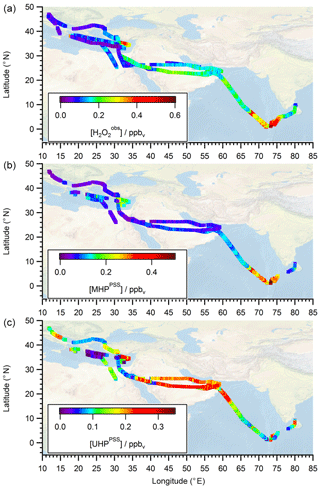Figure 8All flight positions in the upper troposphere (p<300 hPa) during OMO as a function of (a) ${\mathrm{H}}_{\mathrm{2}}{{\mathrm{O}}_{\mathrm{2}}}^{\mathrm{obs}}$, (b) MHPPSS , and (c) UHPPSS.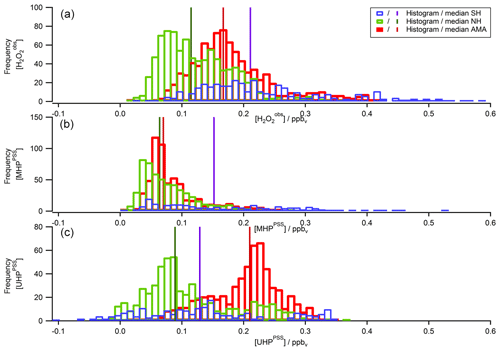Figure 9Histograms of ${\mathrm{H}}_{\mathrm{2}}{{\mathrm{O}}_{\mathrm{2}}}^{\mathrm{obs}}$ (a), MHPPSS (b), and UHPPSS (c) mixing ratios during the OMO campaign for NH background (green), SH (blue), and AMA (red) air masses.

In the analysis of Flight 17 we found a strong correlation between UHPPSS and acetone (Fig. 6) and an increase in UHPPSS at the highest air mass ages, represented by low [NO]  [NOy] (Fig. 7). Extension of this analysis to all observations in the upper troposphere obtained during OMO yields similar results for the relation between UHPPSS, ROOHobs, and ${\mathrm{H}}_{\mathrm{2}}{{\mathrm{O}}_{\mathrm{2}}}^{\mathrm{obs}}$ and acetone (Fig. 10). Enhanced mixing ratios of hydroperoxides are typically associated with enhanced acetone mixing ratios, especially for UHPPSS. A simple calculation of the production of MHP out of the photolysis of acetone and the reaction of acetaldehyde (from EMAC) with OH shows that per day approximately 40 pptv MHP can be formed within the AMA. The lifetime of MHP was calculated to be around 1.5 d. Thus not all of the UHPPSS in the AMA (median 210 pptv) can be accounted for MHP that was chemically produced from VOCs in the AMA. The scatterplots of the hydroperoxides vs. [NO]  [NOy] for the whole data set show no clear correlation with a large spread of hydroperoxide mixing ratios at the lowest [NO]  [NOy] ratios, representing the oldest, i.e., chemically most processed, air masses (Fig. 11).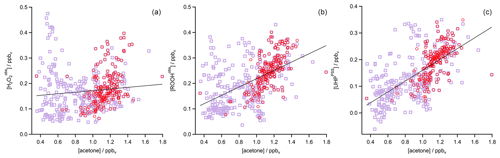Figure 10Scatterplots of acetone and ${\mathrm{H}}_{\mathrm{2}}{{\mathrm{O}}_{\mathrm{2}}}^{\mathrm{obs}}$ (a), ROOHobs (b), and UHPPSS (c) in the UT (purple squares) and especially in the AMA (red circles). The black lines represent the least-orthogonal-distance fit with linear regression coefficients R2 of 0.01 (${\mathrm{H}}_{\mathrm{2}}{{\mathrm{O}}_{\mathrm{2}}}^{\mathrm{obs}}$), 0.27 (ROOHobs), and 0.41 (UHPPSS).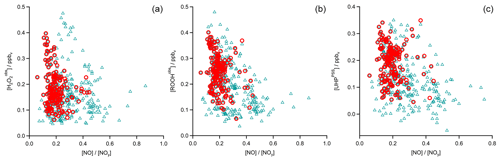Figure 11Scatterplots of NO∕NOy and ${\mathrm{H}}_{\mathrm{2}}{{\mathrm{O}}_{\mathrm{2}}}^{\mathrm{obs}}$ (a), ROOHobs (b), and UHPPSS (c) in the UT (blue triangles) and especially in the AMA (red circles).

A scatterplot of the results from the ${\mathrm{H}}_{\mathrm{2}}{{\mathrm{O}}_{\mathrm{2}}}^{\mathrm{PSS}}$ based on observed HOx data in the UT (Eq. 15) is shown in Fig. 12. The dotted black line shows the 1 : 1 line; the dashed green lines represent the 2 : 1 and 1 : 2 relations. It is obvious that the comparison is affected by a rather large offset of approximately 350 pptv in the observations that is not accounted for in the steady-state calculations. The regression coefficient R2 is 0.26. Most of the ${\mathrm{H}}_{\mathrm{2}}{{\mathrm{O}}_{\mathrm{2}}}^{\mathrm{PSS}}$ mixing ratios (75 %) vary between 0 and 65 pptv, with a median value of 15 pptv, while the ${\mathrm{H}}_{\mathrm{2}}{{\mathrm{O}}_{\mathrm{2}}}^{\mathrm{obs}}$ mixing ratios extend over a larger range, mainly between 10 and 210 pptv, with a median of 150 pptv and thus 10 times higher than for steady-state, indicating that more than 80 % of all points in the correlation are outside the range of uncertainty. This can also be seen in the histograms in Fig. 13. Table 1 shows the statistical comparison of both data sets. The discrepancy between ${\mathrm{H}}_{\mathrm{2}}{{\mathrm{O}}_{\mathrm{2}}}^{\mathrm{obs}}$ and ${\mathrm{H}}_{\mathrm{2}}{{\mathrm{O}}_{\mathrm{2}}}^{\mathrm{PSS}}$ shows that the local PSS does not account for all main contributions of H2O2 even though all chemical reactions are included. Thus transport phenomena like deep convection seem to play a key role (see Sect. 4.3.3).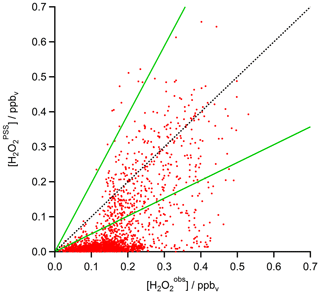Figure 12Scatterplot of ${\mathrm{H}}_{\mathrm{2}}{{\mathrm{O}}_{\mathrm{2}}}^{\mathrm{obs}}$ and ${\mathrm{H}}_{\mathrm{2}}{{\mathrm{O}}_{\mathrm{2}}}^{\mathrm{PSS}}$ mixing ratios (red) with the 1 : 1 (black), 1 : 2, and 2 : 1 (both green) lines.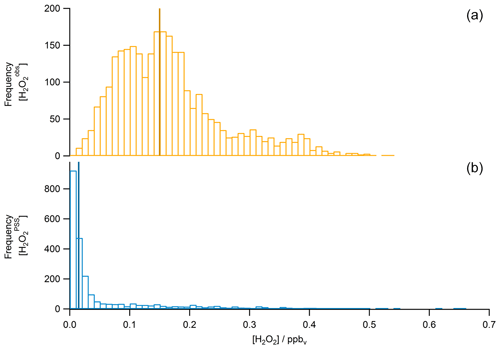Figure 13Histograms of ${\mathrm{H}}_{\mathrm{2}}{{\mathrm{O}}_{\mathrm{2}}}^{\mathrm{obs}}$ (a) and ${\mathrm{H}}_{\mathrm{2}}{{\mathrm{O}}_{\mathrm{2}}}^{\mathrm{PSS}}$ (b) mixing ratios (bars) and the associated medians (lines).

Table 1Comparison of H2O2 mixing ratios in the upper troposphere from observations and PSS calculations.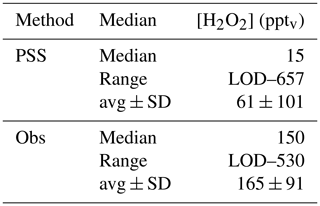### 4.3.2 Comparison to EMAC

Figure 14 shows histograms for the comparison between ${\mathrm{H}}_{\mathrm{2}}{{\mathrm{O}}_{\mathrm{2}}}^{\mathrm{obs}}$, MHPPSS, and UHPPSS with EMAC simulations. Median ${\mathrm{H}}_{\mathrm{2}}{{\mathrm{O}}_{\mathrm{2}}}^{\mathrm{EMAC}}$ values are similar for NH background (66 pptv) and AMA (71 pptv) conditions (Table 2), while ${\mathrm{H}}_{\mathrm{2}}{{\mathrm{O}}_{\mathrm{2}}}^{\mathrm{obs}}$ indicate an enhancement of +64 pptv in the AMA relative to the NH background. For the SH the model-simulated ${\mathrm{H}}_{\mathrm{2}}{{\mathrm{O}}_{\mathrm{2}}}^{\mathrm{EMAC}}$ (272 pptv) mixing ratios are 4 times higher than in the NH background (66 pptv), while the ${\mathrm{H}}_{\mathrm{2}}{{\mathrm{O}}_{\mathrm{2}}}^{\mathrm{obs}}$ mixing ratios only show a median increase by roughly a factor of 2 (100 to 211 pptv; Table 2).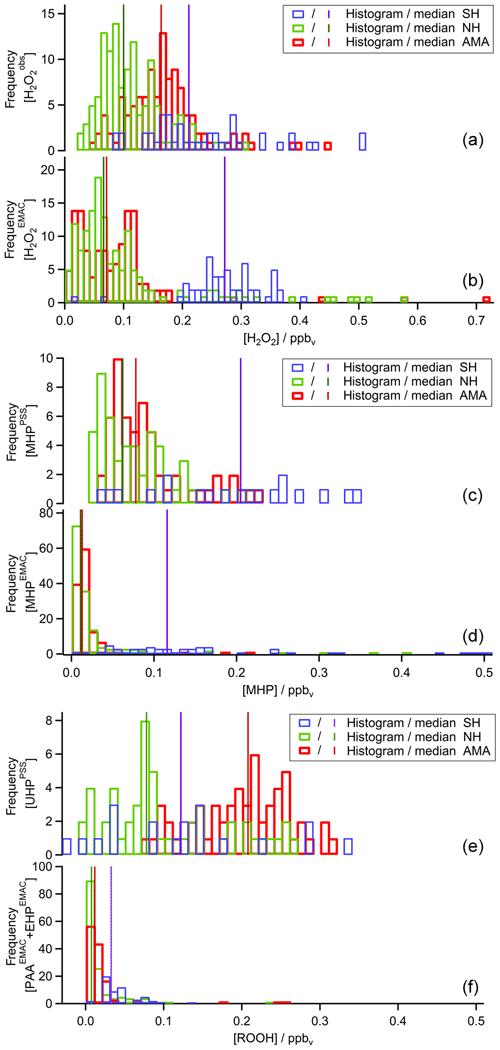Figure 14Histograms of ${\mathrm{H}}_{\mathrm{2}}{{\mathrm{O}}_{\mathrm{2}}}^{\mathrm{obs}}$ and ${\mathrm{H}}_{\mathrm{2}}{{\mathrm{O}}_{\mathrm{2}}}^{\mathrm{EMAC}}$ (a, b), MHPPSS and MHPEMAC (c, d), and UHPPSS and UHPEMAC (given as PAAEMAC and EHPEMAC; e, f) mixing ratios during the OMO campaign for NH background (green), SH (blue), and AMA (red) air masses.

Table 2Comparison of H2O2, MHP and UHP mixing ratios in the upper troposphere from EMAC, measurements and PSS calculations.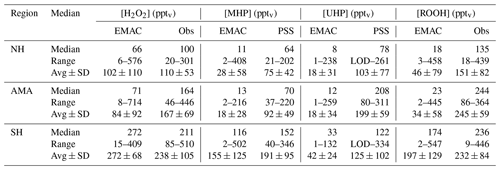In general EMAC tends to strongly underestimate total hydroperoxide in all air masses by a factor of 5–10. MHPEMAC mixing ratios are mainly lower than 50 pptv for background and AMA, while MHPPSS ranges from LOD–140 pptv. Again the model simulates the highest MHPEMAC mixing ratios in the SH, with values up to 502 pptv compared to up to 346 pptv in the MHPPSS calculations. Similar as for ${\mathrm{H}}_{\mathrm{2}}{{\mathrm{O}}_{\mathrm{2}}}^{\mathrm{EMAC}}$, medians of MHPEMAC for NH background and AMA conditions show very small differences (11 and 13 pptv, respectively; Table 2), while slightly higher differences were found for UHPEMAC in the AMA (64 and 70 pptv, respectively). In the simulations, southern hemispheric MHPEMAC mixing ratios are almost 10 times higher than NH background values (116 and 11 pptv, respectively) compared to 2 to 3 times higher in the observations.

Data for UHPEMAC in the model are calculated from the sum of simulated ethyl hydroperoxide (EHP) and peroxyacetic acid (PAA), which, according to the model, are the only nonmethyl organic hydroperoxides in the free troposphere with nonzero mixing ratios. UHPEMAC mixing ratios range from 1 to 238 pptv in the NH background, 1 to 259 pptv in the AMA, and 1 to 132 pptv in the SH. UHPPSS based on the observations indicate lowest mixing ratios in the NH background (LOD–261 pptv), while in the AMA and the SH the ranges are quite similar (80–311 pptv and LOD–334 pptv). A comparison of median values emphasizes the large difference between model simulations and observation-based estimates. In the NH background, the median UHPPSS mixing ratio from the observations is 70 pptv higher than EMAC simulations (78 and 8 pptv, respectively). In the AMA the difference is even larger, with about 200 pptv higher UHPPSS levels compared to the EMAC simulations. The smallest difference, with 89 pptv, was found for the SH (Table 2).

So far discussions of different air masses have been based on measurements of methane, subdividing the observations into NH background, AMA, and SH data. Tomsche et al. (2019) have shown that longitudinal gradients are found in the AMA over the Arabian Peninsula. Observations in the west are often near the edge of the anticyclone, while observations towards the east are closer to its center. In Fig. 16, observations, steady-state calculations, and EMAC simulations for upper tropospheric (9–15 km) H2O2 are displayed as a function of longitude from west to east (20–30 N, 36–60 E; according to the red box in Fig. 15). To identify gradients, the data are subdivided into bins of 2 longitude. The observations (orange) show roughly a 100 % increase in ${\mathrm{H}}_{\mathrm{2}}{{\mathrm{O}}_{\mathrm{2}}}^{\mathrm{obs}}$ from west to east (90 to 175 pptv), similar to simulation with EMAC (black), although absolute mixing ratio levels in ${\mathrm{H}}_{\mathrm{2}}{{\mathrm{O}}_{\mathrm{2}}}^{\mathrm{EMAC}}$ are smaller (61 to 121 pptv). Contrary to these observed gradients, ${\mathrm{H}}_{\mathrm{2}}{{\mathrm{O}}_{\mathrm{2}}}^{\mathrm{PSS}}$ mixing ratios based on HORUS data (blue) do not vary with longitude, except for the last two bins. The steady-state calculations are based exclusively on observed concentrations of HO2 and OH radicals and thus yield only the net photochemical production, while the EMAC simulations and the observations will also account for vertical and horizontal advection from upwind source regions. Previous studies show inconsistent results. Snow et al. (2007) and Barth et al. (2016) for example both show that H2O2 is depleted in convective outflow compared to background upper troposphere. In contrast, other studies found that deep convection can be a source of H2O2 in the upper troposphere (e.g., Jaeglé et al., 1997; Prather and Jacob, 1997; Mari et al., 2003; Bozem et al., 2017). Similarly, convection over India during the summer monsoon is a potential source of excess H2O2 in the upper troposphere. With a photochemical lifetime of several days, this excess in H2O2 reaches the western AMA, giving rise to the observed and model-simulated longitudinal gradients. Since the steady-state calculations do not account for transport, this can explain the rather large deviation of 144–164 pptv (between 51 and 57) from the observations. Differences between observation and EMAC simulation could potentially arise due to uncertainties in the scavenging efficiency for H2O2 as the chemistry does not seem to be a dominant cause of uncertainty.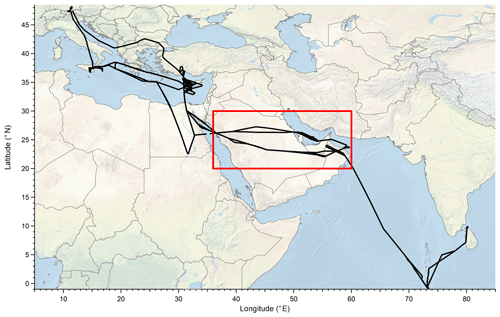Figure 15Location of measurements used for the longitudinal gradient study (red box) out of all flight tracks (black).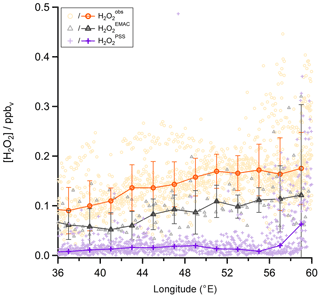Figure 16Longitudinal trends of ${\mathrm{H}}_{\mathrm{2}}{{\mathrm{O}}_{\mathrm{2}}}^{\mathrm{obs}}$ mixing ratios (orange circles), ${\mathrm{H}}_{\mathrm{2}}{{\mathrm{O}}_{\mathrm{2}}}^{\mathrm{EMAC}}$ (black triangles), and ${\mathrm{H}}_{\mathrm{2}}{{\mathrm{O}}_{\mathrm{2}}}^{\mathrm{PSS}}$ (purple plus signs). The data are shown in the light colors while the darker ones represent the medians.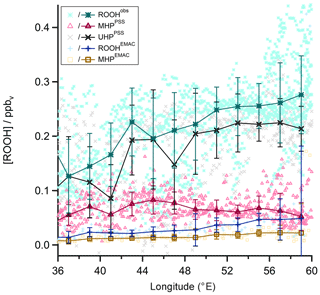Figure 17Longitudinal trends of ROOHobs mixing ratios (green asterisks), ROOHEMAC (blue plus signs), and mixing ratios for MHPPSS (pink triangles) and UHPPSS (black crosses) as well as MHPEMAC (yellow squares). The data are shown in the light colors, while the darker ones represent the medians.

Similar longitudinal gradients are also observed for measured total organic hydroperoxides (ROOHobs; green asterisks in Fig. 17), inferred UHPPSS (black); and total ROOHEMAC (blue). Steady-state calculations of MHPPSS (pink) and simulations of MHPEMAC (yellow) show either no or only weak longitudinal gradients. Assuming that MHP is also enhanced in the outflow of deep convection (Mari et al., 2000; Barth et al., 2016), at least part of the enhancement in ROOHobs (and thus inferred UHPPSS) could be due to advected MHP.

## 4.4 Discussion

To our knowledge we present the first observations of H2O2 and ROOH mixing ratios in the Asian monsoon anticyclone. Previous studies have been mainly focused on the northern hemispheric upper troposphere. Several aircraft campaigns including peroxide measurements were performed over North America. They are summarized in Snow et al., 2007): the SONEX campaign took place in fall 1997 in the UT and yielded mean values of 120 pptv for H2O2 and 50 pptv for MHP (medians: 80 and 30 pptv, respectively). The TOPSE campaign in winter/spring 2000 probed the middle troposphere, yielding median H2O2 and MHP mixing ratios of 150 pptv for both species. During the INTEX-NA campaign in summer 2004, observed median mixing ratios at altitudes of 6–10 km were about 400 pptv for H2O2 and 200 pptv for MHP. A comparison with our results (Table 2) shows that we found similar mixing ratios as in SONEX in the northern hemispheric background of 115 and 64 pptv for H2O2 and MHP, respectively. Mixing ratios for both species reported for TOPSE and INTEX-NA are slightly higher than ours, which may be related to the lower altitude range of 6–10 km (in comparison to >9 km for OMO) in these studies. Previous observations have shown that H2O2 and MHP show the highest mixing ratios at altitudes between 2 and 5 km followed by a sharp decrease towards higher altitudes (see e.g., Daum et al., 1990; Heikes, 1992; Weinstein-Lloyd et al., 1998; Snow, 2003; Snow et al., 2007; Klippel et al., 2011).

Heikes et al. (1996) associated enhanced H2O2 mixing ratios above 5 km in the North Pacific of the Asian coast (30 N) with outflow from Typhoon Mireille (Heikes et al., 1996). These observations were made close to the source region for the AMA-influenced air masses described here (see back trajectories in the case study of Flight 17; Fig. 2 or Tomsche et al., 2019). For MHP Heikes et al. (1996) found mixing ratios of 250–500 pptv in the southern longitudinal section above 5 km, similar to median mixing ratios of 152 pptv for MHP in SH air masses in the UT found in this study.

Although the mixing ratios observed during this study are similar to previous observations in the upper troposphere, one striking result is that a state-of-the-art global circulation model (EMAC) and a local steady-state calculation constrained by measured radical levels significantly underestimate H2O2 mixing ratios in particular in the AMA. The general tendency is that the steady-state model produces the lowest values, with EMAC falling in between steady state and observations (e.g., Fig. 16). A comparison of the EMAC simulations for the two radicals that affect H2O2 most strongly (OH and HO2) yields a rather good agreement. A scatterplot between modeled and observed HO2 yields a slope of 0.72±0.01 (pptv pptv−1) and an offset of 4.30±0.09 pptv, with a regression coefficient R2 of 0.58 (Fig. 18 left). The OH data show more scatter, with a tendency for EMAC to overestimate the mixing ratios (slope: 1.7±0.2 (pptv pptv−1); offset: $-\mathrm{0.1}±\mathrm{0.1}$ pptv; regression coefficient R2: 0.09; Fig. 18 right). Although there is rather good agreement between EMAC simulations and observations for all the species that affect the local photochemical budget of H2O2, EMAC significantly exceeds PSS calculation for H2O2. This is an indication that an additional H2O2 source is accounted for in the global model and that the local photostationary-state assumption is not fulfilled. The additional source is attributed to transport associated with deep convection over India, yielding an upwind source of H2O2 that is significant throughout the western part of the AMA. In the AMA, clouds are absent so that gas-phase photochemical processes may determine the lifetime of H2O2. Based on observed OHobs levels and photolysis frequencies during OMO, the H2O2 lifetime in the upper troposphere is of the order of several days, sufficiently long for the excess H2O2 to reach the western parts of the AMA, producing the observed longitudinal H2O2 gradient observed in both observations and EMAC simulations (Fig. 16). The total amount of H2O2 injected into the UT by convective outflow depends on the scavenging efficiency (Mari et al., 2000; Barth et al., 2016; Bozem et al., 2017). Differences between ${\mathrm{H}}_{\mathrm{2}}{{\mathrm{O}}_{\mathrm{2}}}^{\mathrm{obs}}$ and ${\mathrm{H}}_{\mathrm{2}}{{\mathrm{O}}_{\mathrm{2}}}^{\mathrm{EMAC}}$ are most likely due to an overestimation of scavenging in the model as also pointed out by Klippel et al., 2011).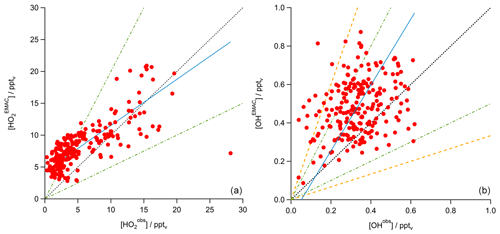Figure 18Scatterplot of ${{\mathrm{HO}}_{\mathrm{2}}}^{\mathrm{obs}}$ and ${{\mathrm{HO}}_{\mathrm{2}}}^{\mathrm{EMAC}}$ data (a) and OHobs and OHEMAC data (b; both red) with the 1 : 1 (black), 1 : 2, and 2 : 1 (both green) lines. The blue line shows the calculated least-orthogonal-distance fit.

To investigate this assumption we performed a sensitivity study with the wet scavenging for all soluble species being switched off globally. The result is shown in Fig. 19. The ${\mathrm{H}}_{\mathrm{2}}{{\mathrm{O}}_{\mathrm{2}}}^{\mathrm{EMAC}}$ mixing ratios significantly increase with longitude by a factor of 3–4 and thus to the level of ${\mathrm{H}}_{\mathrm{2}}{{\mathrm{O}}_{\mathrm{2}}}^{\mathrm{obs}}$. Please note that significant enhancements in MHPEMAC and ROOHEMAC were not found in the sensitivity study with switched-off scavenging, indicating that the strong underestimation by the model of these species is not due to an overestimation of wet removal in convective clouds. Instead, we found that EMAC underestimates ROOH in all air masses and not only in the AMA. The reasons for this underestimation are unknown. In a previous comparison of MHP observations and EMAC simulations over Europe for July 2007, Klippel et al. (2011) also reported a difference of a factor of 10 in the upper troposphere, while a comparison during the fall season (October 2006) yielded a rather good agreement (within a factor of 2).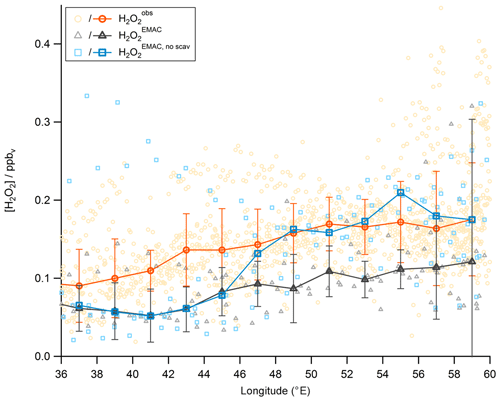Figure 19Longitudinal trends of ${\mathrm{H}}_{\mathrm{2}}{{\mathrm{O}}_{\mathrm{2}}}^{\mathrm{obs}}$ mixing ratios (orange circles), ${\mathrm{H}}_{\mathrm{2}}{{\mathrm{O}}_{\mathrm{2}}}^{\mathrm{EMAC}}$ (black triangles), and the sensitivity study without scavenging in ${\mathrm{H}}_{\mathrm{2}}{{\mathrm{O}}_{\mathrm{2}}}^{\mathrm{EMAC}}$ (blue circles). The data are shown in the light colors, while the darker ones represent the medians.

There is a rather large uncertainty regarding the scavenging efficiency of MHP in deep convection (Barth et al., 2016). For the Trace A campaign Mari et al. (2000) found observed (modeled) enhancement ratios of postconvective mixing ratios in comparison to preconvective mixing ratios of 11 (9.5) for MHP and 1.9 (1.2) for H2O2. Such efficient transport in the Indian summer monsoon would yield a strong source of upper-tropospheric MHP, explaining the large enhancement of ROOHobs in the AMA described here. Please note that large enhancements of MHPEMAC and ROOHEMAC were not found in the sensitivity study with switched-off scavenging, indicating that the strong underestimation by the model of those species is not due to an overestimation of wet removal in convective clouds. It seems that a large part of the UHPPSS is actually MHP advected throughout the AMA after deep convective transport over India. In the EMAC simulations the transport of MHP is less efficient, and thus MHPEMAC is lower than MHPPSS and UHPPSS. Please note that EMAC has a general tendency to overestimate CO in the UT, especially for the NH background, while it tends to underestimate CH4 (Tomsche et al., 2019). The deviations in general are not significant, with the exception of CH4 in the AMA (Table 1 in Tomsche et al., 2019). As discussed in Tomsche et al. (2019), the CH4 mixing ratio in the AMA depends of the colocation of convection and underlying methane sources. The model resolution of 2.8× 2.8 is not sufficient to resolve small-scale variations in both convection and CH4 source distribution. The H2O2 mixing ratio over the Indian subcontinent is not expected to show large spatial variations since latitudinal gradients are generally small (see, e.g., Klippel et al., 2011). Therefore, we do not expect that the model resolution will have a strong influence on the deviation between ${\mathrm{H}}_{\mathrm{2}}{{\mathrm{O}}_{\mathrm{2}}}^{\mathrm{obs}}$ and ${\mathrm{H}}_{\mathrm{2}}{{\mathrm{O}}_{\mathrm{2}}}^{\mathrm{EMAC}}$. Another uncertainty arises from missing information on the absolute mixing ratios of H2O2, MHP, and higher organic hydroperoxides in the inflow region of deep convection over India since observations of these species in the boundary layer over India are not available. Note that the amount of hydrogen peroxide and number of organic hydroperoxides transported to the upper troposphere depends on the scavenging efficiency and on the mixing ratios of the individual species in the inflow region (Barth et al., 2016; Bozem et al., 2017). Thus, an underestimation of hydroperoxides in the upper troposphere after convective injection can be due either to an underestimation of the scavenging efficiency for individual species, an underestimation of their mixing ratio in the inflow region, or a combination of both and might differ for individual hydroperoxides. Due to a lack of observations in the inflow and outflow region of convection over India, this question cannot be resolved in this study.

5 Conclusions

Hydrogen peroxide and organic hydroperoxides were measured during the OMO campaign in the upper troposphere in NH background air over the western Mediterranean, the Asian summer monsoon anticyclone over the Arabian Peninsula, and the SH over the Maldives and the Indian Ocean in summer 2015. The observed mixing ratios for background conditions in the NH and SH are in line with previous studies described in the literature. A case study (of Flight 17) revealed enhanced ${\mathrm{H}}_{\mathrm{2}}{{\mathrm{O}}_{\mathrm{2}}}^{\mathrm{obs}}$ and ROOHobs mixing ratios in the AMA relative to the NH background. Similar results are found for other flights throughout the campaign. The atmospheric chemistry general circulation model EMAC slightly underestimates ${\mathrm{H}}_{\mathrm{2}}{{\mathrm{O}}_{\mathrm{2}}}^{\mathrm{EMAC}}$ in the NH background (medians: 66 pptv vs. 100 pptv), significantly underestimates it in the AMA (medians: 71 pptv vs. 164 pptv), and overestimates it in the SH (medians: 272 pptv vs. 211 pptv). Steady-state calculations for ${\mathrm{H}}_{\mathrm{2}}{{\mathrm{O}}_{\mathrm{2}}}^{\mathrm{PSS}}$ and MHPPSS based on observed precursors yield much lower values compared to ${\mathrm{H}}_{\mathrm{2}}{{\mathrm{O}}_{\mathrm{2}}}^{\mathrm{obs}}$ and MHPPSS by roughly a factor of 3, in particular in the AMA, resulting in a large contribution of an unidentified organic hydroperoxide (UHPPSS) in air masses affected by the AMA. A comparison between EMAC simulations and HOx levels shows a good agreement, indicating that deviations between ${\mathrm{H}}_{\mathrm{2}}{{\mathrm{O}}_{\mathrm{2}}}^{\mathrm{EMAC}}$ and ${\mathrm{H}}_{\mathrm{2}}{{\mathrm{O}}_{\mathrm{2}}}^{\mathrm{PSS}}$ levels are due to transport. Convective injection of H2O2 (and ROOH) into the upper troposphere over India most likely forms a pool of hydroperoxides in the upper troposphere that subsequently influences the western AMA, giving rise to a significant longitudinal gradient of H2O2 and ROOH mixing ratios, with increasing values towards the center of the AMA. It is likely that next to an unidentified organic hydroperoxide (e.g., PAA), at least part of UHPPSS is due to additional MHP from an upwind source. A sensitivity study using EMAC with no scavenging tends to reproduce the observed longitudinal gradients in H2O2, although it does not increase the level of ROOH. The reasons for this different behavior are unclear.

Data availability

The data are available from the HALO database (https://halo-db.pa.op.dlr.de/mission/0, OMO, 2015).

Author contributions

BH and SH were responsible for H2O2 and ROOH measurements and data. BH conducted further data analysis and wrote the original draft of the paper in close cooperation with HF. CH4 and CO data were provided by LT; HOx data by DM, MM, and HH; O3 and acetone data by MN and AZ; photolysis frequencies by BB and NO; and NOy data by HZ and GS. AP was responsible for the EMAC model simulations. JL was the principal investigator of the OMO mission. All authors were involved in the review and editing of the paper.

Competing interests

The authors declare that they have no conflict of interest.

Acknowledgements

We would like to thank all of the participants of the OMO mission, the German Aerospace Center (DLR), and EDT Offshore Ltd in Cyprus for their cooperation during the mission. We further thank Rainer Königstedt for installing the TRIHOP instrument and Uwe Parchatka for supporting the measurements of CO and CH4.

Financial support

The article processing charges for this open-access publication were covered by the Max Planck Society.

Review statement

This paper was edited by Barbara Ervens and reviewed by two anonymous referees.

References

AEROLASER: AL2021 H2O2-Monitor User Manual, Version 2.20, Rev.02, Garmisch-Partenkirchen, Germany, 2006.

Atkinson, R., Baulch, D. L., Cox, R. A., Crowley, J. N., Hampson, R. F., Hynes, R. G., Jenkin, M. E., Rossi, M. J., and Troe, J.: Evaluated kinetic and photochemical data for atmospheric chemistry: Volume I – gas phase reactions of Ox, HOx, NOx and SOx species, Atmos. Chem. Phys., 4, 1461–1738, https://doi.org/10.5194/acp-4-1461-2004, 2004.

Atkinson, R., Baulch, D. L., Cox, R. A., Crowley, J. N., Hampson, R. F., Hynes, R. G., Jenkin, M. E., Rossi, M. J., Troe, J., and IUPAC Subcommittee: Evaluated kinetic and photochemical data for atmospheric chemistry: Volume II – gas phase reactions of organic species, Atmos. Chem. Phys., 6, 3625–4055, https://doi.org/10.5194/acp-6-3625-2006, 2006.

Barret, B., Sauvage, B., Bennouna, Y., and Le Flochmoen, E.: Upper-tropospheric CO and O3 budget during the Asian summer monsoon, Atmos. Chem. Phys., 16, 9129–9147, https://doi.org/10.5194/acp-16-9129-2016, 2016.

Barth, M. C., Bela, M. M., Fried, A., Wennberg, P. O., Crounse, J. D., St. Clair, J. M., Blake, N. J., Blake, D. R., Homeyer, C. R., Brune, W. H., Zhang, L., Mao, J., Ren, X., Ryerson, T. B., Pollack, I. B., Peischl, J., Cohen, R. C., Nault, B. A., Huey, L. G., Liu, X., and Cantrell, C. A.: Convective transport and scavenging of peroxides by thunderstorms observed over the central U.S. during DC3, J. Geophys. Res.-Atmos., 121, 4272–4295, https://doi.org/10.1002/2015JD024570, 2016.

Bohn, B. and Lohse, I.: Calibration and evaluation of CCD spectroradiometers for ground-based and airborne measurements of spectral actinic flux densities, Atmos. Meas. Tech., 10, 3151–3174, https://doi.org/10.5194/amt-10-3151-2017, 2017.

Bozem, H., Pozzer, A., Harder, H., Martinez, M., Williams, J., Lelieveld, J., and Fischer, H.: The influence of deep convection on HCHO and H2O2 in the upper troposphere over Europe, Atmos. Chem. Phys., 17, 11835–11848, https://doi.org/10.5194/acp-17-11835-2017, 2017.

Burkholder, J. B., Sander, S. P., Abbatt, J., Barker, J. R., Huie, R. E., Kolb, C. E., Kurylo, M. J., Orkin, V. L., Wilmouth, D. M., and Wine, P. H.: Chemical Kinetics and Photochemical Data for Use in Atmospheric Studies, JPL Publication 15-10, Jet Propulsion Laboratory, Pasadena, 2015.

Calvert, J. G., Lazrus, A., Kok, G. L., Heikes, B. G., Walega, J. G., Lind, J., and Cantrell, C. A.: Chemical mechanisms of acid generation in the troposphere, Nature, 317, 27–35, https://doi.org/10.1038/317027a0, 1985.

Crutzen, P. J., Lawrence, M. G., and Pöschl, U.: On the background photochemistry of tropospheric ozone, Tellus B, 51, 123–146, https://doi.org/10.3402/tellusb.v51i1.16264, 1999.

Daum, P. H., Kleinman, L. I., Hills, A. J., Lazrus, A. L., Leslie, A. C. D., Busness, K., and Boatman, J.: Measurement and interpretation of concentrations of H2O2 and related species in the upper midwest during summer, J. Geophys. Res., 95, 9857–9871, https://doi.org/10.1029/JD095iD07p09857, 1990.

Emanuel, K. A. and Zivkovic-Rothman, M.: Development and Evaluation of a Convection Scheme for Use in Climate Models, J. Atmos. Sci., 1766–1782, https://doi.org/10.1175/1520-0469(1999)056<1766:DAEOAC>2.0.CO;2, 1999.

Faloona, I., Tan, D., Brune, W. H., Jaeglé, L., Jacob, D. J., Kondo, Y., Koike, M., Chatfield, R., Pueschel, R., Ferry, G., Sachse, G., Vay, S., Anderson, B., Hannon, J., and Fuelberg, H.: Observations of HOx and its relationship with NOx in the upper troposphere during SONEX, J. Geophys. Res., 105, 3771–3783, https://doi.org/10.1029/1999JD900914, 2000.

Faloona, I. C., Tan, D., Lesher, R. L., Hazen, N. L., Frame, C. L., Simpas, J. B., Harder, H., Martinez, M., Di Carlo, P., Ren, X., and Brune, W. H.: A Laser-induced Fluorescence Instrument for Detecting Tropospheric OH and HO2: Characteristics and Calibration, J. Atmos. Chem., 47, 139–167, https://doi.org/10.1023/B:JOCH.0000021036.53185.0e, 2004.

Gettelman, A., Kinnison, D. E., Dunkerton, T. J., and Brasseur, G. P.: Impact of monsoon circulations on the upper troposphere and lower stratosphere, J. Geophys. Res., 109, D22101, https://doi.org/10.1029/2004JD004878, 2004.

Heikes, B. G.: Formaldehyde and hydroperoxides at Mauna Loa Observatory, J. Geophys. Res., 97, 18001, https://doi.org/10.1029/92JD00268, 1992.

Heikes, B. G., Lee, M., Bradshaw, J., Sandholm, S., Davis, D. D., Crawford, J., Rodriguez, J., Liu, S., McKeen, S., Thornton, D., Bandy, A., Gregory, G., Talbot, R., and Blake, D.: Hydrogen peroxide and methylhydroperoxide distributions related to ozone and odd hydrogen over the North Pacific in the fall of 1991, J. Geophys. Res., 101, 1891–1905, https://doi.org/10.1029/95JD01364, 1996.

Hoffmann, M. R. and Edwards, J. O.: Kinetics of the oxidation of sulfite by hydrogen peroxide in acidic solution, J. Phys. Chem., 79, 2096–2098, https://doi.org/10.1021/j100587a005, 1975.

Jackson, A. V. and Hewitt, C. N.: Hydrogen peroxide and organic hydroperoxide concentrations in air in a eucalyptus forest in central Portugal, Atmos. Environ., 30, 819–830, https://doi.org/10.1016/1352-2310(95)00348-7, 1996.

Jacob, P. and Klockow, D.: Hydrogen peroxide measurements in the marine atmosphere, J. Atmos. Chem., 15, 353–360, https://doi.org/10.1007/BF00115404, 1992.

Jaeglé, L., Jacob, D. J., Wennberg, P. O., Spivakovsky, C. M., Hanisco, T. F., Lanzendorf, E. J., Hintsa, E. J., Fahey, D. W., Keim, E. R., Proffitt, M. H., Atlas, E. L., Flocke, F., Schauffler, S., McElroy, C. T., Midwinter, C., Pfister, L., and Wilson, J. C.: Observed OH and HO2 in the upper troposphere suggest a major source from convective injection of peroxides, Geophys. Res. Lett., 24, 3181–3184, https://doi.org/10.1029/97GL03004, 1997.

Jaeglé, L., Jacob, D. J., Brune, W. H., Faloona, I., Tan, D., Heikes, B. G., Kondo, Y., Sachse, G. W., Anderson, B., Gregory, G. L., Singh, H. B., Pueschel, R., Ferry, G., Blake, D. R., and Shetter, R. E.: Photochemistry of HOx in the upper troposphere at northern midlatitudes, J. Geophys. Res., 105, 3877–3892, https://doi.org/10.1029/1999JD901016, 2000.

Jöckel, P., Tost, H., Pozzer, A., Kunze, M., Kirner, O., Brenninkmeijer, C. A. M., Brinkop, S., Cai, D. S., Dyroff, C., Eckstein, J., Frank, F., Garny, H., Gottschaldt, K.-D., Graf, P., Grewe, V., Kerkweg, A., Kern, B., Matthes, S., Mertens, M., Meul, S., Neumaier, M., Nützel, M., Oberländer-Hayn, S., Ruhnke, R., Runde, T., Sander, R., Scharffe, D., and Zahn, A.: Earth System Chemistry integrated Modelling (ESCiMo) with the Modular Earth Submodel System (MESSy) version 2.51, Geosci. Model Dev., 9, 1153–1200, https://doi.org/10.5194/gmd-9-1153-2016, 2016.

Klippel, T., Fischer, H., Bozem, H., Lawrence, M. G., Butler, T., Jöckel, P., Tost, H., Martinez, M., Harder, H., Regelin, E., Sander, R., Schiller, C. L., Stickler, A., and Lelieveld, J.: Distribution of hydrogen peroxide and formaldehyde over Central Europe during the HOOVER project, Atmos. Chem. Phys., 11, 4391-4410, https://doi.org/10.5194/acp-11-4391-2011, 2011.

Lawrence, M. G. and Lelieveld, J.: Atmospheric pollutant outflow from southern Asia: a review, Atmos. Chem. Phys., 10, 11017–11096, https://doi.org/10.5194/acp-10-11017-2010, 2010.

Lazrus, A. L., Kok, G. L., Gitlin, S. N., Lind, J. A., and McLaren, S. E.: Automated fluorimetric method for hydrogen peroxide in atmospheric precipitation, Anal. Chem., 57, 917–922, https://doi.org/10.1021/ac00281a031, 1985.

Lazrus, A. L., Kok, G. L., Lind, J. A., Gitlin, S. N., Heikes, B. G., and Shetter, R. E.: Automated fluorometric method for hydrogen peroxide in air, Anal. Chem., 58, 594–597, https://doi.org/10.1021/ac00294a024, 1986.

Lee, M., Heikes, B. G., and O'Sullivan, D. W.: Hydrogen peroxide and organic hydroperoxide in the troposphere: A review, Atmos. Environ., 34, 3475–3494, https://doi.org/10.1016/S1352-2310(99)00432-X, 2000.

Lelieveld, J. and Crutzen, P. J.: Influences of cloud photochemical processes on tropospheric ozone, Nature, 343, 227–233, https://doi.org/10.1038/343227a0, 1990.

Lelieveld, J., Berresheim, H., Borrmann, S., Crutzen, P. J., Dentener, F. J., Fischer, H., Feichter, J., Flatau, P. J., Heland, J., Holzinger, R., Korrmann, R., Lawrence, M. G., Levin, Z., Markowicz, K. M., Mihalopoulos, N., Minikin, A., Ramanathan, V., Reus, M. de, Roelofs, G. J., Scheeren, H. A., Sciare, J., Schlager, H., Schultz, M., Siegmund, P., Steil, B., Stephanou, E. G., Stier, P., Traub, M., Warneke, C., Williams, J., and Ziereis, H.: Global air pollution crossroads over the Mediterranean, Science, 298, 794–799, https://doi.org/10.1126/science.1075457, 2002.

Lelieveld, J., Bourtsoukidis, E., Brühl, C., Fischer, H., Fuchs, H., Harder, H., Hofzumahaus, A., Holland, F., Marno, D., Neumaier, M., Pozzer, A., Schlager, H., Williams, J., Zahn, A., and Ziereis, H.: The South Asian monsoon-pollution pump and purifier, Science, 361, 270–273, https://doi.org/10.1126/science.aar2501, 2018.

Levy, H.: Normal atmosphere: large radical and formaldehyde concentrations predicted, Science, 173, 141–143, https://doi.org/10.1126/science.173.3992.141, 1971.

Lind, J. A. and Kok, G. L.: Henry's law determinations for aqueous solutions of hydrogen peroxide, methylhydroperoxide, and peroxyacetic acid, J. Geophys. Res., 91, 7889–7895, https://doi.org/10.1029/JD091iD07p07889, 1986.

Lind, J. A. and Kok, G. L.: Correction to “Henry's law determinations for aqueous solutions of hydrogen peroxide, methylhydroperoxide, and peroxyacetic acid”, J. Geophys. Res., 99, 21119, https://doi.org/10.1029/94JD01155, 1994.

Mari, C., Jacob, D. J., and Bechtold, P.: Transport and scavenging of soluble gases in a deep convective cloud, J. Geophys. Res., 105, 22255–22267, https://doi.org/10.1029/2000JD900211, 2000.

Mari, C., Saüt, C., Jacob, D. J., Staudt, A., Avery, M. A., Brune, W. H., Faloona, I., Heikes, B. G., Sachse, G. W., Sandholm, S. T., Singh, H. B., and Tan, D.: On the relative role of convection, chemistry, and transport over the South Pacific Convergence Zone during PEM-Tropics B: A case study, J. Geophys. Res., 108, 401, https://doi.org/10.1029/2001JD001466, 2003.

Martinez, M., Harder, H., Kubistin, D., Rudolf, M., Bozem, H., Eerdekens, G., Fischer, H., Klüpfel, T., Gurk, C., Königstedt, R., Parchatka, U., Schiller, C. L., Stickler, A., Williams, J., and Lelieveld, J.: Hydroxyl radicals in the tropical troposphere over the Suriname rainforest: airborne measurements, Atmos. Chem. Phys., 10, 3759–3773, https://doi.org/10.5194/acp-10-3759-2010, 2010.

Nunnermacker, L. J., Weinstein-Lloyd, J. B., Hillery, B., Giebel, B., Kleinman, L. I., Springston, S. R., Daum, P. H., Gaffney, J., Marley, N., and Huey, G.: Aircraft and ground-based measurements of hydroperoxides during the 2006 MILAGRO field campaign, Atmos. Chem. Phys., 8, 7619–7636, https://doi.org/10.5194/acp-8-7619-2008, 2008.

Ojha, N., Pozzer, A., Rauthe-Schöch, A., Baker, A. K., Yoon, J., Brenninkmeijer, C. A. M., and Lelieveld, J.: Ozone and carbon monoxide over India during the summer monsoon: regional emissions and transport, Atmos. Chem. Phys., 16, 3013–3032, https://doi.org/10.5194/acp-16-3013-2016, 2016.

OMO (Oxidation Mechanism Observations): Oxidation Mechanism Observations in the extrattropical free TS, HALO database, available at: https://halo-db.pa.op.dlr.de/mission/0 (last access: 7 November 2019), 2015.

Penkett, S. A., Jones, B., Brich, K. A., and Eggleton, A.: The importance of atmospheric ozone and hydrogen peroxide in oxidising sulphur dioxide in cloud and rainwater, Atmos. Environ., 13, 123–137, https://doi.org/10.1016/0004-6981(79)90251-8, 1979.

Perros, P.: Large-scale distribution of hydrogen peroxide from aircraft measurements during the TROPOZ II experiment, Atmos. Environ. A-Gen., 27, 1695–1708, https://doi.org/10.1016/0960-1686(93)90232-N, 1993.

Pilz, W. and Johann, I.: Die Bestimmung Kleinster Mengen von Wasserstoffperoxyd in Luft, Int. J Environ. Anal. Chem., 3, 257–270, https://doi.org/10.1080/03067317408071087, 1974.

Prather, M. J. and Jacob, D. J.: A persistent imbalance in HOx and NOx photochemistry of the upper troposphere driven by deep tropical convection, Geophys. Res. Lett., 24, 3189–3192, https://doi.org/10.1029/97GL03027, 1997.

Randel, W. J., Park, M., Emmons, L., Kinnison, D., Bernath, P., Walker, K. A., Boone, C., and Pumphrey, H.: Asian monsoon transport of pollution to the stratosphere, Science, 328, 611–613, https://doi.org/10.1126/science.1182274, 2010.

Rauthe-Schöch, A., Baker, A. K., Schuck, T. J., Brenninkmeijer, C. A. M., Zahn, A., Hermann, M., Stratmann, G., Ziereis, H., van Velthoven, P. F. J., and Lelieveld, J.: Trapping, chemistry, and export of trace gases in the South Asian summer monsoon observed during CARIBIC flights in 2008, Atmos. Chem. Phys., 16, 3609–3629, https://doi.org/10.5194/acp-16-3609-2016, 2016.

Robbin Martin, L. and Damschen, D. E.: Aqueous oxidation of sulfur dioxide by hydrogen peroxide at low pH, Atmos. Environ., 15, 1615–1621, https://doi.org/10.1016/0004-6981(81)90146-3, 1981.

Roeckner, E., Brokopf, R., Esch, M., Giorgetta, M., Hagemann, S., Kornblueh, L., Manzini, E., Schlese, U., and Schulzweida, U.: Sensitivity of Simulated Climate to Horizontal and Vertical Resolution in the ECHAM5 Atmosphere Model, J. Climate, 19, 3771–3791, https://doi.org/10.1175/JCLI3824.1, 2006.

Schiller, C. L., Bozem, H., Gurk, C., Parchatka, U., Königstedt, R., Harris, G. W., Lelieveld, J., and Fischer, H.: Applications of quantum cascade lasers for sensitive trace gas measurements of CO, CH4, N2O and HCHO, Appl. Phys. B, 92, 419–430, https://doi.org/10.1007/s00340-008-3125-0, 2008.

Slemr, F. and Tremmel, H. G.: Hydroperoxides in the marine troposphere over the Atlantic Ocean, J. Atmos. Chem., 19, 371–404, https://doi.org/10.1007/BF00694493, 1994.

Snow, J. A.: Winter-spring evolution and variability of HOx reservoir species, hydrogen peroxide, and methyl hydroperoxide, in the northern middle to high latitudes, J. Geophys. Res., 108, 1890, https://doi.org/10.1029/2002JD002172, 2003.

Snow, J. A., Heikes, B. G., Shen, H., O'Sullivan, D. W., Fried, A., and Walega, J.: Hydrogen peroxide, methyl hydroperoxide, and formaldehyde over North America and the North Atlantic, J. Geophys. Res., 112, 8353, https://doi.org/10.1029/2006JD007746, 2007.

Stohl, A., Forster, C., Frank, A., Seibert, P., and Wotawa, G.: Technical note: The Lagrangian particle dispersion model FLEXPART version 6.2, Atmos. Chem. Phys., 5, 2461–2474, https://doi.org/10.5194/acp-5-2461-2005, 2005.

Stratmann, G., Ziereis, H., Stock, P., Brenninkmeijer, C., Zahn, A., Rauthe-Schöch, A., Velthoven, P. V., Schlager, H., and Volz-Thomas, A.: NO and NOy in the upper troposphere: Nine years of CARIBIC measurements onboard a passenger aircraft, Atmos. Environ., 133, 93–111, https://doi.org/10.1016/j.atmosenv.2016.02.035, 2016.

Tadic, I., Parchatka, U., Königstedt, R., and Fischer, H.: In-flight stability of quantum cascade laser-based infrared absorption spectroscopy measurements of atmospheric carbon monoxide, Appl. Phys. B, 123, 805, https://doi.org/10.1007/s00340-017-6721-z, 2017.

Tomsche, L., Pozzer, A., Ojha, N., Parchatka, U., Lelieveld, J., and Fischer, H.: Upper tropospheric CH4 and CO affected by the South Asian summer monsoon during the Oxidation Mechanism Observations mission, Atmos. Chem. Phys., 19, 1915–1939, https://doi.org/10.5194/acp-19-1915-2019, 2019.

Weinstein-Lloyd, J. B., Lee, J. H., Daum, P. H., Kleinman, L. I., Nunnermacker, L. J., Springston, S. R., and Newman, L.: Measurements of peroxides and related species during the 1995 summer intensive of the Southern Oxidants Study in Nashville, Tennessee, J. Geophys. Res., 103, 22361–22373, https://doi.org/10.1029/98JD01636, 1998.

Zahn, A., Weppner, J., Widmann, H., Schlote-Holubek, K., Burger, B., Kühner, T., and Franke, H.: A fast and precise chemiluminescence ozone detector for eddy flux and airborne application, Atmos. Meas. Tech., 5, 363–375, https://doi.org/10.5194/amt-5-363-2012, 2012.

Ziereis, H., Schlager, H., Schulte, P., van Velthoven, P. F. J., and Slemr, F.: Distributions of NO, NOx and NOy in the upper troposphere and lower stratosphere between 28 and 61 N during POLINAT 2, J. Geophys. Res., 105, 3653–3664, https://doi.org/10.1029/1999JD900870, 2000.